Courses
Courses for Kids
Free study material
Offline Centres
More

# NCERT Exemplar for Class 11 Chemistry Chapter-12 (Book Solutions)Last updated date: 27th Nov 2023
Total views: 610.5k
Views today: 12.10k

## NCERT Exemplar for Class 11 Chemistry - Organic Chemistry - Some Basic Principles and Techniques

Chapter 12 of Class 11 Chemistry, Organic Chemistry, introduces the basics of the fascinating world of Organic Chemistry to the students. This chapter forms the basics of organic chemistry which are very crucial for Class 12, students will be able to understand all the important concepts required for Class 12 from the NCERT exemplar questions of Chapter 12. Students can download free PDFs of the solutions to all the NCERT exemplar questions given in this chapter from the official website of Vedantu, which they can access at any time.

Free PDF download of NCERT Exemplar for Class 11 Chemistry Chapter 12 - Organic Chemistry - Some Basic Principles and Techniques solved by expert Chemistry teachers on Vedantu.com as per NCERT (CBSE) Book guidelines. All Chapter 12 - Organic Chemistry - Some Basic Principles and Techniques exercise questions with solutions to help you to revise the complete syllabus and score more marks in your examinations.

## Access NCERT Exemplar Solutions for Class 11 Chemistry Chapter 12 - Organic Chemistry - Some Basic Principles and Techniques

### Multiple Choice Questions (Type-I)

1. Which of the following is the correct IUPAC name?

(i) 3-Ethyl-4, 4-dimethylheptane

(ii) 4,4-Dimethyl-3-ethylheptane

(iii) 5-Ethyl-4, 4-dimethylheptane

(iv) 4,4-Bis(methyl)-3-ethylheptane

Ans: Option (i) 3-Ethyl-4, 4-dimethylheptane is correct since in IUPAC naming the different alkyl groups present are written in alphabetical order. Di, tri, tetra are not included in the alphabetical order. So, “e” of the ethyl group comes before the “m” of the dimethyl group. The number 3 is given to the ethyl group and is written first.

2. The IUPAC name for $\text{C}{{\text{H}}_{\text{3}}}\text{0}\overset{\begin{matrix} \text{ }\!\!~\!\!\text{ O} \\ || \\\end{matrix}}{\mathop{\text{C}}}\,\text{-C}{{\text{H}}_{\text{3}}}\text{-C}{{\text{H}}_{\text{3}}}\text{0}\overset{\begin{matrix} \text{ }\!\!~\!\!\text{ O} \\ \text{ }\!\!~\!\!\text{ }\!\!|\!\!\text{ }\!\!|\!\!\text{ } \\\end{matrix}}{\mathop{\text{C}}}\,\text{-OH}$ is ________

.

(i) 1-hydroxypentane-1,4-dione

(ii) 1,4-dioxopentanol

(iii) 1-carboxybutan-3-one

(iv) 4-oxopentanoic acid

Ans: Option (iv) 4-oxopentanoic acid is correct  since more than one functional group is present in the chain so, out of the two functional groups one will be given more priority as a result numbering is done from that side and mentioned as a sec. suffix, while the other one is written as prefix and mentioned as oxo along with the locant.

3. The IUPAC name for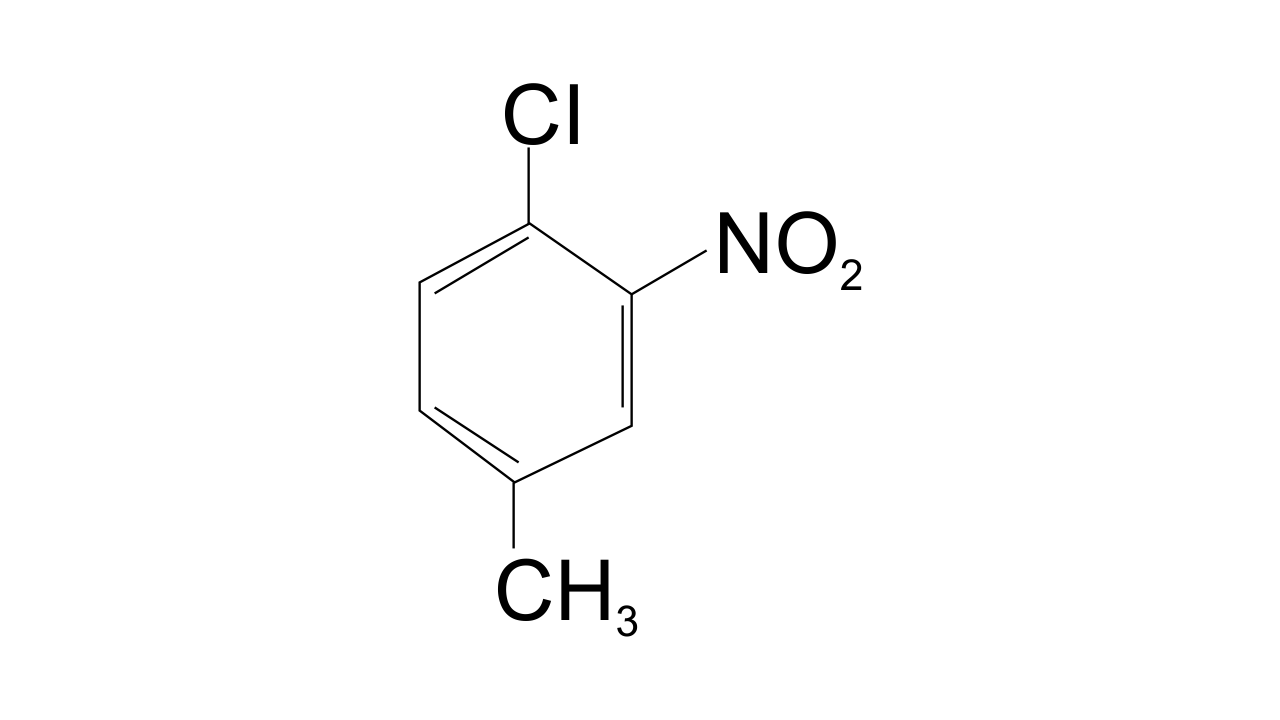(i) 1-Chloro-2-nitro-4-methylbenzene

(ii) 1-Chloro-4-methyl-2-nitrobenzene

(iii) 2-Chloro-1-nitro-5-methylbenzene

(iv) m-Nitro-p-chlorotoluene

Ans: Option (ii) 1-Chloro-4-methyl-2-nitrobenzene is correct since the substituent of the base compound is given number 1 and then the direction of numbering is chosen in such a manner that the next substituent gets the lowest number. The substituents that appear in the IUPAC name are in alphabetical order.  Hence in this case numbering can be shown as-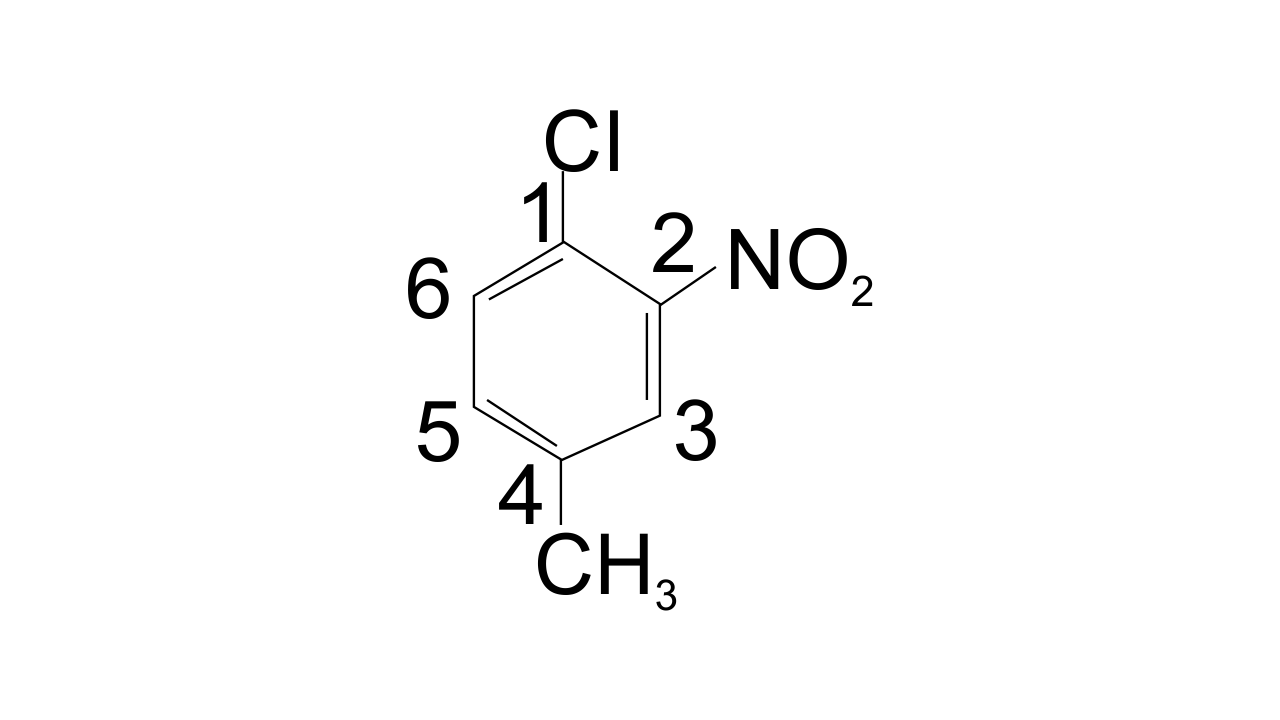4. Electronegativity of carbon atoms depends upon their state of hybridisation. In which of the following compounds, the carbon marked with asterisk is most electronegative?

$\begin{array}{*{35}{l}} \left( \mathbf{i} \right)\text{ }\mathbf{C}{{\mathbf{H}}_{\mathbf{3}}}\text{ }\text{ }\mathbf{C}{{\mathbf{H}}_{\mathbf{2}}}\text{ }\text{ }*\mathbf{C}{{\mathbf{H}}_{\mathbf{2}}}\text{ }\mathbf{C}{{\mathbf{H}}_{\mathbf{3}}} \\ \left( \mathbf{ii} \right)\text{ }\mathbf{C}{{\mathbf{H}}_{\mathbf{3}}}\text{ }\text{ }*\mathbf{CH}\text{ }=\text{ }\mathbf{CH}\text{ }\text{ }\mathbf{C}{{\mathbf{H}}_{\mathbf{3}}} \\ \left( \mathbf{iii} \right)\text{ }\mathbf{C}{{\mathbf{H}}_{\mathbf{3}}}\text{ }\text{ }\mathbf{C}{{\mathbf{H}}_{\mathbf{2}}}\text{ }\text{ }\mathbf{C}\text{ }\equiv \text{ }*\mathbf{CH} \\ \left( \mathbf{iv} \right)\text{ }\mathbf{C}{{\mathbf{H}}_{\mathbf{3}}}\text{ }\text{ }\mathbf{C}{{\mathbf{H}}_{\mathbf{2}}}\text{ }\text{ }\mathbf{CH}\text{ }=\text{ }*\mathbf{C}{{\mathbf{H}}_{\mathbf{2}}} \\\end{array}$

Ans: Option (iii) $\text{C}{{\text{H}}_{\text{3}}}\text{ -- C}{{\text{H}}_{\text{2}}}\text{ -- C }\equiv \text{ *CH}$ is correct since electronegativity of carbon depends on its hybridized state and percentage of s-character. Electronegativity $\propto$ percentage of  s-character.

So, the order of percentage ‘s’ character is given as-

$\text{s}{{\text{p}}^{\text{3}}}$ (25%) < $\text{s}{{\text{p}}^{2}}$ (33%) < $\text{sp}$ (50%)

In option (iii) Carbon linked through triple bond and is sp hybridized hence it is highly electronegative.

5. In which of the following, functional group isomerism is not possible?

(i) Alcohols

(ii) Aldehydes

(iii) Alkyl halides

(iv) Cyanides

Ans: Option (iii) Alkyl halides is correct since functional isomers are the ones which have the same molecular formula but different functional groups being present in them.

Alcohols are functional isomers of ethers while aldehydes are the functional isomers of ketones whereas cyanides are functional isomers of isocyanides.  Only alkyl halides are the ones which do not show functional isomerism.

6. The fragrance of flowers is due to the presence of some steam volatile organic compounds called essential oils. These are generally insoluble in water at room temperature but are miscible with water vapour in vapour phase. A suitable method for the extraction of these oils from the flowers is:

(i) Distillation

(ii) Crystallisation

(iii) Distillation under reduced pressure

(iv) Steam distillation

Ans: Option (iv) Steam distillation is correct since this technique is applicable to separate the substances which are steam volatile and are immiscible with water. In steam distillation, steam from a generator is passed through a heated flask containing the liquid to be distilled. The mixture of steam and the volatile organic compound is then condensed and is collected. The compound is later separated from water using the separating funnel.

7. During hearing of a court case, the judge suspected that some changes in the documents had been carried out. He asked the forensic department to check the ink used at two different places. According to you which technique can give the best results?

(i) Column chromatography

(ii) Solvent extraction

(iii) Distillation

(iv) Thin layer chromatography

Ans: Option (iv) Thin layer chromatography is correct since thin layer chromatography is a method for analysing the mixtures by separating the components of the mixture. This technique can be used to determine the number of components in a mixture, the identity of compounds and the purity of compounds by observing the appearance of a product or the disappearance of a reactant. It can also be used to analyse the progress of a reaction.

8. The principle involved in paper chromatography is

(ii) Partition

(iii) Solubility

(iv) Volatility

Ans: Option (ii) Partition is correct since partition chromatography is based on the principle of continuous differential partitioning of components of a mixture between a stationary and a mobile phase. Paper chromatography is a type of partition chromatography only.

9. What is the correct order of decreasing stability of the following cations?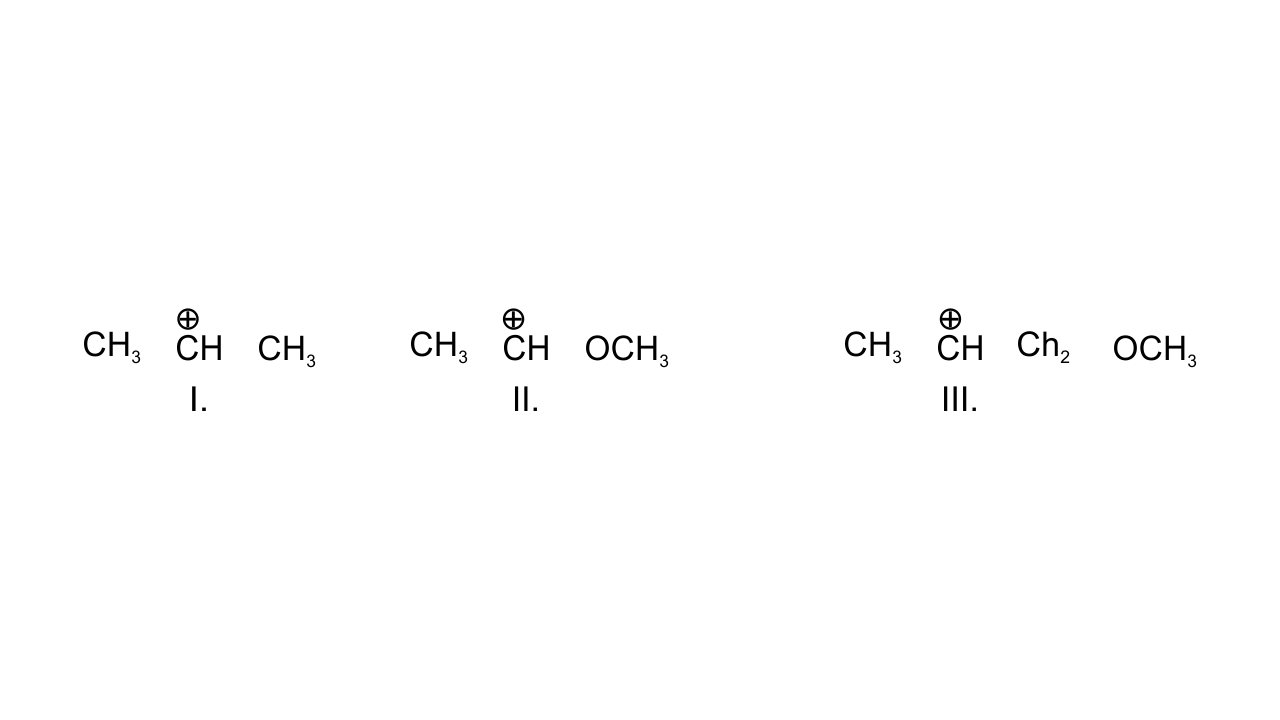(i) II > I > III

(ii) II > III > I

(iii) III > I > II

(iv) I > II > III

Ans:  Option (i) II > I > III is correct since in case (I), positively charged C is attached to two alkyl groups and is stabilized through  +I effect. In case (II), +R effect of - $\text{OC}{{\text{H}}_{\text{3}}}$group stabilizes this carbocation. In case (III), - I effect of - $\text{OC}{{\text{H}}_{\text{3}}}$ group destabilizes the carbocation.

Hence, the order of stability is given as:

II > I >III.

10. Correct IUPAC name for is ___________.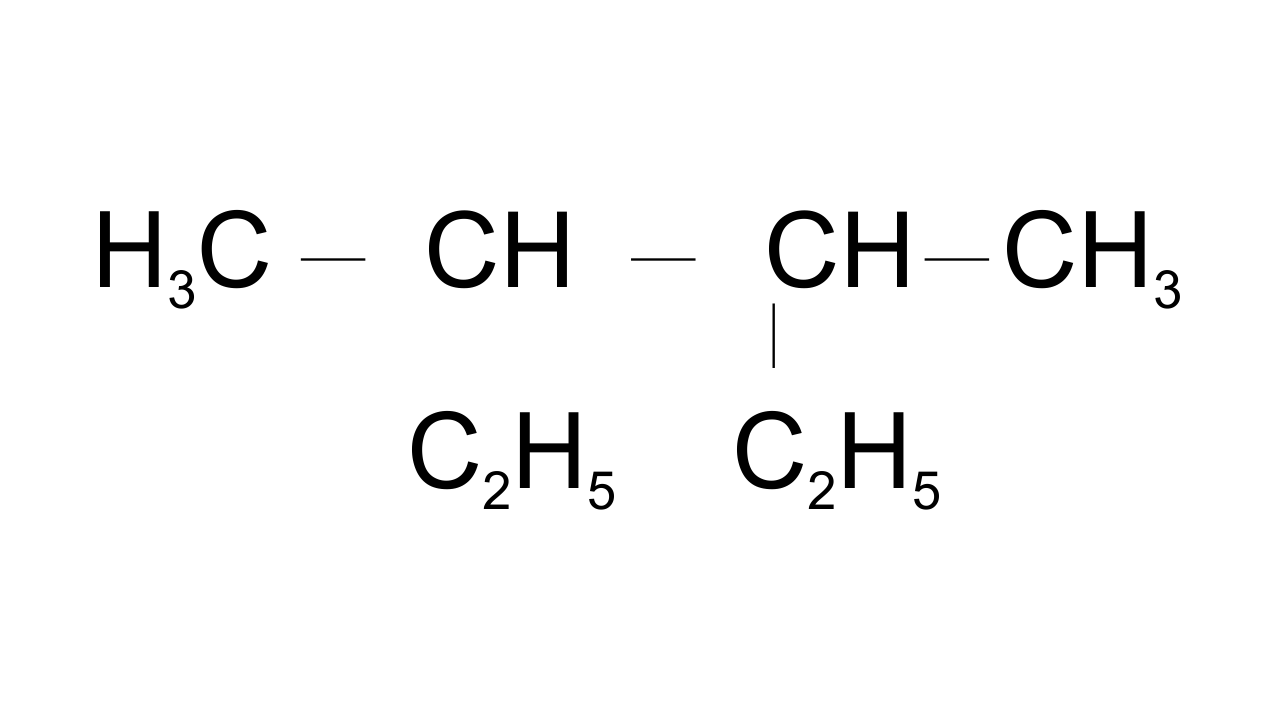(i) 2- ethyl-3-methylpentane

(ii) 3,4- dimethylhexane

(iii) 2-sec-butylbutane

(iv) 2, 3-dimethylbutane

Ans: Option (ii) 3,4- dimethyl hexane is correct since in selecting the longest continuous carbon chain both the ethyl groups are included while the third and fourth carbons have methyl groups as a substituent.

11. In which of the following compounds the carbon marked with asterisk is expected to have greatest positive charge?

$\begin{array}{*{35}{l}} \left( \mathbf{i} \right)\text{ }*\mathbf{C}{{\mathbf{H}}_{\mathbf{3}}}\mathbf{C}{{\mathbf{H}}_{\mathbf{2}}}\text{ }\mathbf{Cl} \\ \left( \mathbf{ii} \right)\text{ }*\mathbf{C}{{\mathbf{H}}_{\mathbf{3}}}\mathbf{C}{{\mathbf{H}}_{\mathbf{2}}}\mathbf{M}{{\mathbf{g}}^{+}}\text{ }\mathbf{C}{{\mathbf{l}}^{}} \\ \left( \mathbf{iii} \right)\text{ }*\mathbf{C}{{\mathbf{H}}_{\mathbf{3}}}\mathbf{C}{{\mathbf{H}}_{\mathbf{2}}}\text{ }\mathbf{Br} \\ \left( \mathbf{iv} \right)\text{ }*\mathbf{C}{{\mathbf{H}}_{\mathbf{3}}}\mathbf{C}{{\mathbf{H}}_{\mathbf{2}}}\mathbf{C}{{\mathbf{H}}_{\mathbf{3}}} \\\end{array}$

Ans: Option (i) is found to be the correct one since the order of electronegativity of the attached group is found to be:

Cl > Br > C > Mg.

Greater the electronegativity of the group attached to the C  greater will be the positive charge. Therefore, in case (i) asterisk C will have the greatest positive charge.

12. Ionic species are stabilised by the dispersal of charge. Which of the following carboxylate ion is the most stable?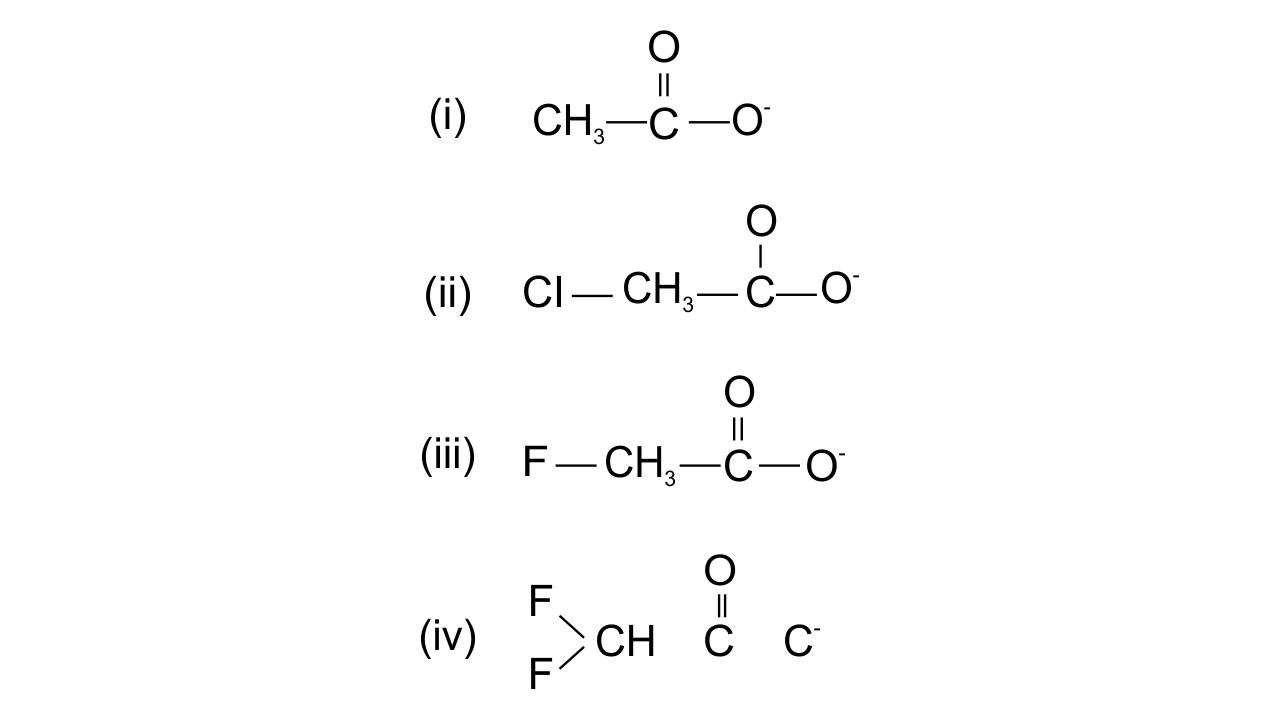Ans: Option (iv) is found to be the correct one since the stabilisation of carboxylate ion depends upon the dispersal of the negative charge. And the dispersal depends upon two factors, i.e., +R effect of the carboxylate ion and Inductive effect of the halogens. In all the above structures, +R effect is common, but halogen atoms are uncommon. Therefore, dispersal of negative charge depends upon the halogen atoms. Fluorine is most electronegative, in structure (iv) two F atoms are present and hence more dispersal of negative charge is there.

13. Electrophilic addition reactions proceed in two steps. The first step involves the addition of an electrophile. Name the type of intermediate formed in the first step of the following addition reaction.

${{\mathbf{H}}_{\mathbf{3}}}\text{ }\mathbf{C}\mathbf{HC}\text{ }=\text{ }\mathbf{C}{{\mathbf{H}}_{\mathbf{2}}}\text{ }+\text{ }{{\mathbf{H}}^{+}}\to ?$

(i) 2° Carbanion

(ii) 1° Carbocation

(iii) 2° Carbocation

(iv) 1° Carbanion

Ans: Option (iii) 2° Carbocation is correct since ${{\text{H}}^{\text{+}}}$attacks on propene and the delocalization of electrons can take place in two possible ways which can be shown as-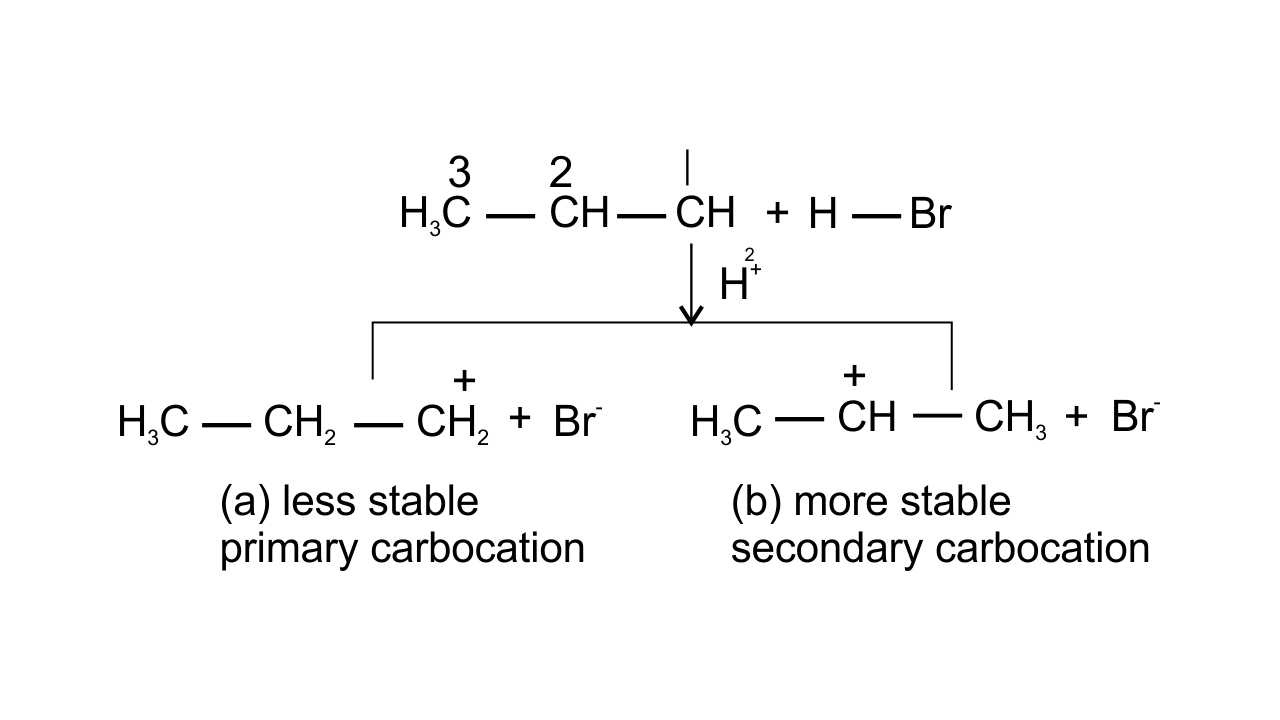14. Covalent bond can undergo fission in two different ways. The correct representation involving a heterolytic fission of $\mathbf{C}{{\mathbf{H}}_{\mathbf{3}}}\mathbf{Br}$ is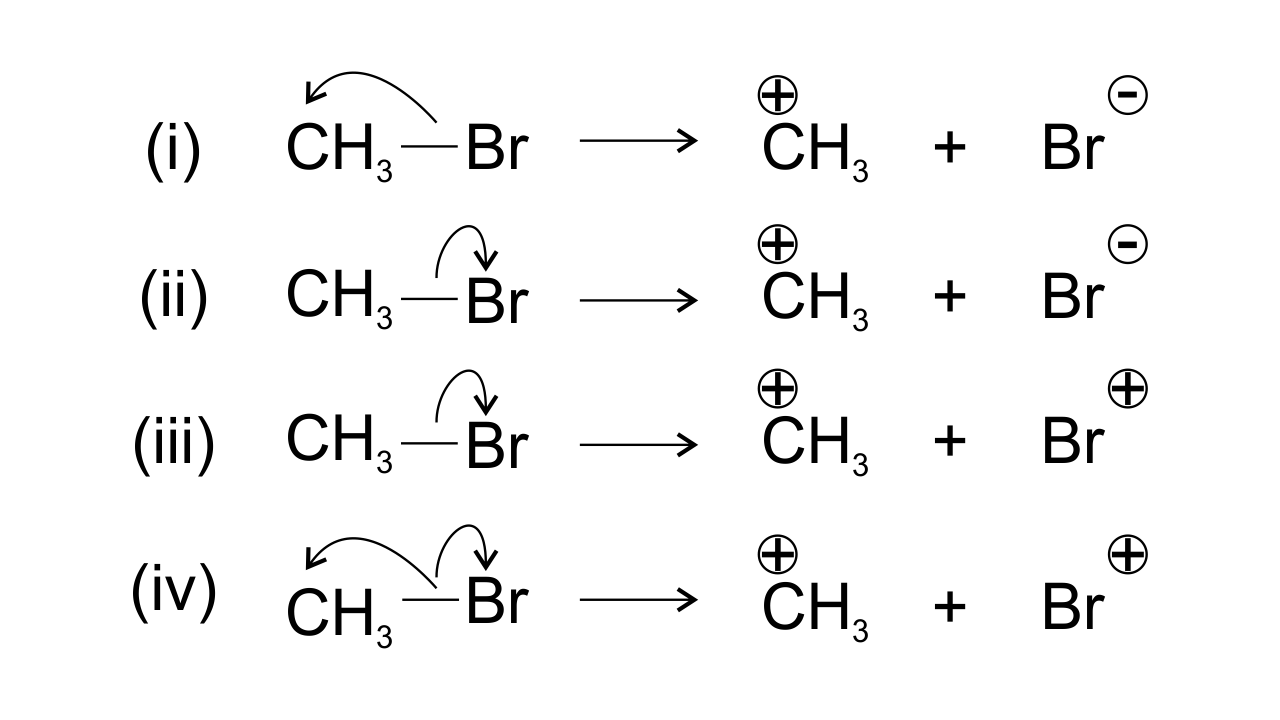Ans: Bromine is found to be more electronegative than Carbon which leads to heterolytic fission. Due to the higher electronegativity the electrons displace from carbon to Br. Therefore, $\text{C}{{\text{H}}_{\text{3}}}$ gets positive charge and Br gets negative charge and hence option (ii) is found to be the correct one.

15. The addition of HCl to an alkene proceeds in two steps. The first step is the attack of ${{\mathbf{H}}^{\mathbf{+}}}$ion to portion which can be shown as-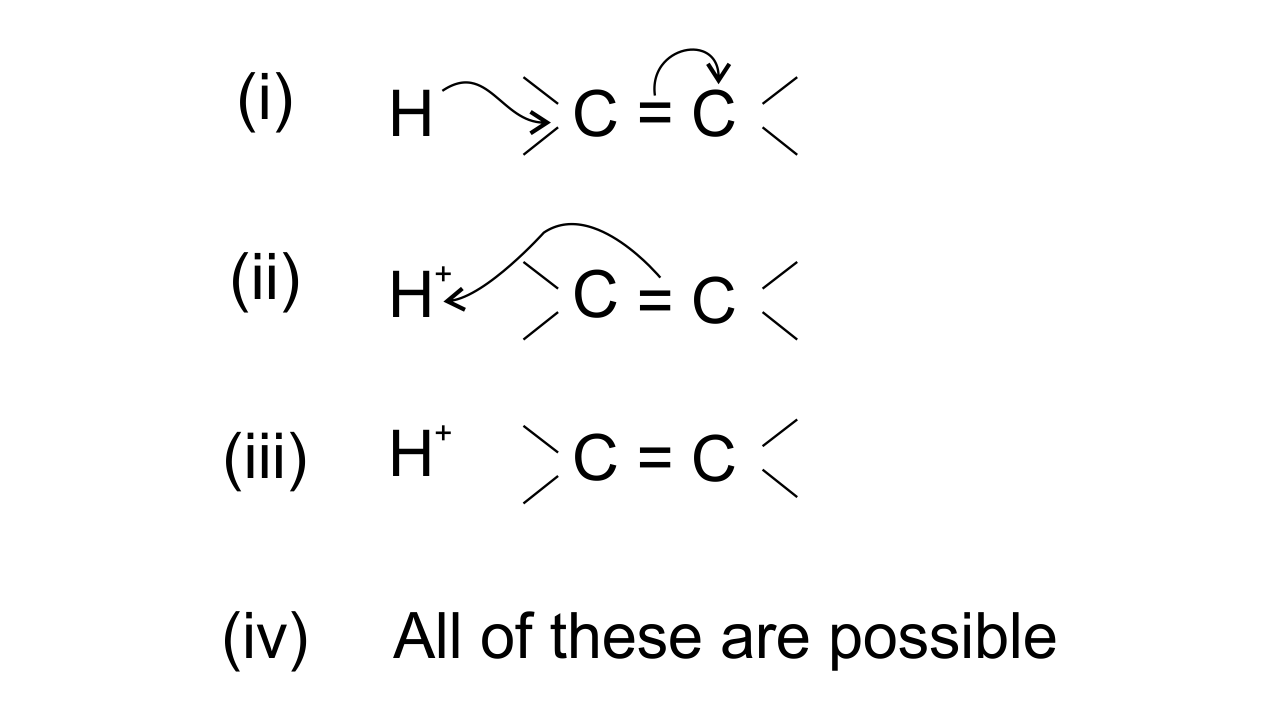Ans: Option (ii) is correct since the arrow shows the direction of electron transfer. In the given reaction, the electron flows from π bond towards the electron-deficient ${{\text{H}}^{\text{+}}}$group . This can be represented as-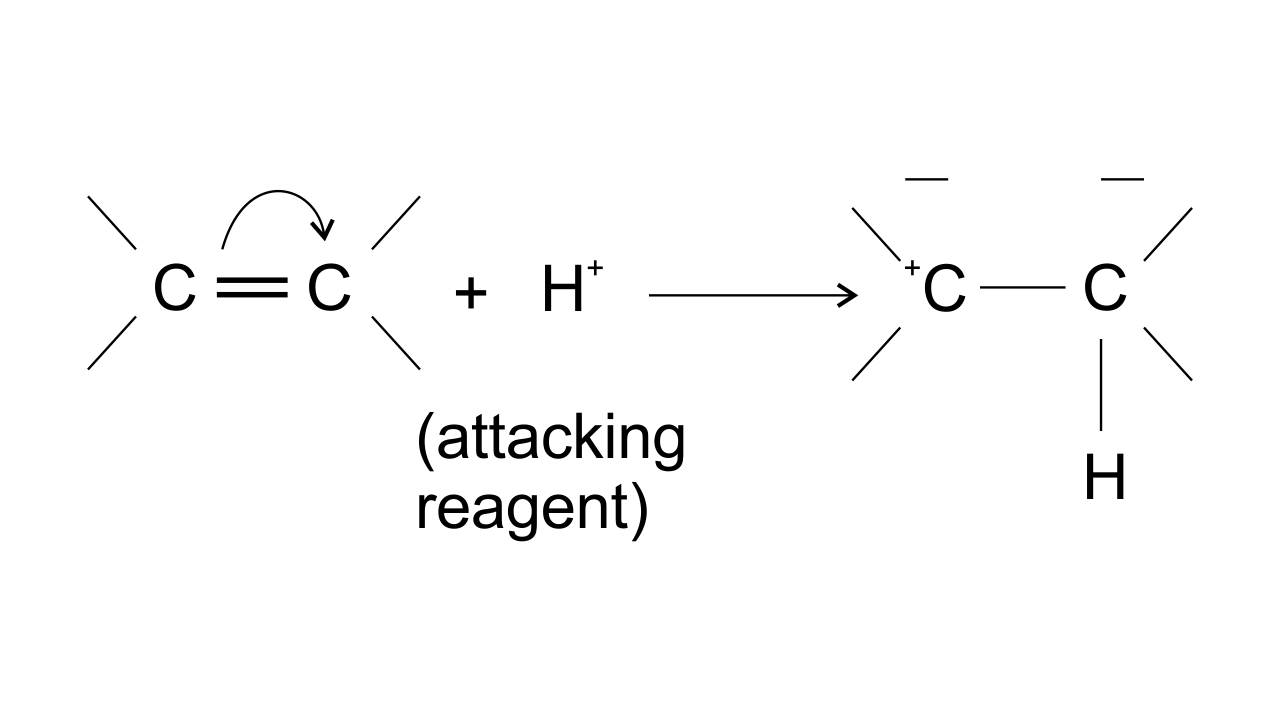### II. Multiple Choice Questions (Type-II)

In the following questions two or more options may be correct.

16. Which of the following compounds contain all the carbon atoms in the same hybridisation state?

$\begin{array}{*{35}{l}} \left( \mathbf{i} \right)\text{ }\mathbf{H}\mathbf{C}\text{ }\equiv \text{ }\mathbf{C}\mathbf{C}\text{ }\equiv \text{ }\mathbf{C}\mathbf{H} \\ \left( \mathbf{ii} \right)\text{ }\mathbf{C}{{\mathbf{H}}_{\mathbf{3}}}\mathbf{C}\text{ }\equiv \text{ }\mathbf{C}\mathbf{C}{{\mathbf{H}}_{\mathbf{3}}} \\ \left( \mathbf{iii} \right)\text{ }\mathbf{C}{{\mathbf{H}}_{\mathbf{2}}}\text{ }=\text{ }\mathbf{C}\text{ }=\text{ }\mathbf{C}{{\mathbf{H}}_{\mathbf{2}}} \\ \left( \mathbf{iv} \right)\text{ }\mathbf{C}{{\mathbf{H}}_{\mathbf{2}}}\text{ }=\text{ }\mathbf{CH}\mathbf{CH}\text{ }=\text{ }\mathbf{C}{{\mathbf{H}}_{\mathbf{2}}} \\\end{array}$

Ans: Options (i) and (iv) are correct since only in these two compounds all the carbon atoms are having same hybridization i.e., sp hybridized in option (i) and $\text{s}{{\text{p}}^{\text{2}}}$ hybridized in option (iv).

17. In which of the following representations given below spatial arrangement of group/ atom different from that given in structure ‘A’?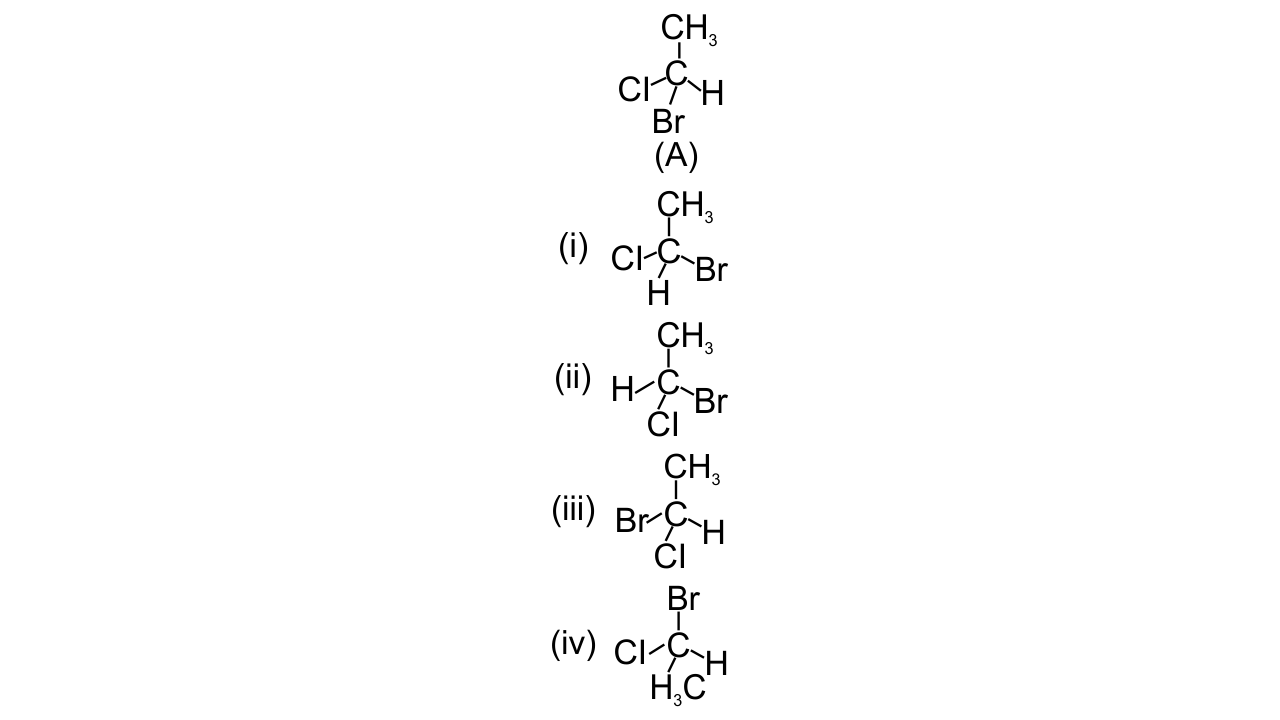Ans: Options (i), (iii) and (iv) are correct since the spatial arrangement of groups or atoms can be checked by doing two interchanges and bringing the H atom below the plane of the paper. Then find out the sequence of the left-out groups in a particular order whether clockwise or anticlockwise starting from the atom with highest atomic number to the atom with lower atomic numbers. So, out of the given options option (ii) has the same spatial arrangement as (A) while in rest three spatial arrangements are different.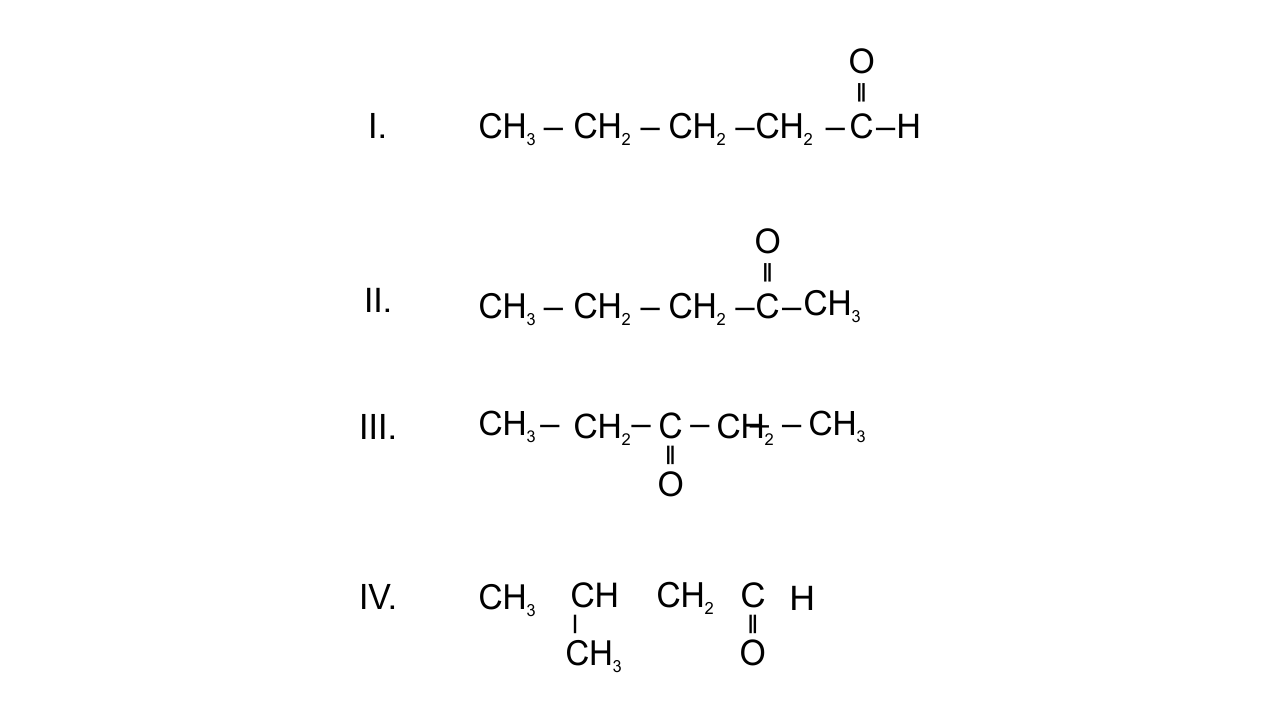18. Electrophiles are electron seeking species. Which of the following groups contain only electrophiles?

${{\begin{array}{*{35}{l}} \left( \mathbf{i} \right)\mathbf{B}{{\mathbf{F}}_{\mathbf{3}}}\mathbf{ , N}{{\mathbf{H}}_{\mathbf{3}}}\mathbf{ , }{{\mathbf{H}}_{\mathbf{2}}}\mathbf{O} \\ \left( \mathbf{ii} \right)\mathbf{AlC}{{\mathbf{l}}_{\mathbf{3}}}\mathbf{, S}{{\mathbf{O}}_{\mathbf{3}}}\mathbf{ , N}{{\mathbf{O}}_{\mathbf{2}}}^{\mathbf{+}} \\ \left( \mathbf{iii} \right)\mathbf{N}{{\mathbf{O}}_{\mathbf{2}}}\mathbf{+ , C}{{\mathbf{H}}_{\mathbf{3}}}^{\mathbf{+}}\mathbf{ , C}{{\mathbf{H}}_{\mathbf{3}}}\mathbf{-}\overset{\mathbf{+}}{\mathop{\mathbf{C}}}\,\mathbf{ = O} \\ \left( \mathbf{iv} \right)\mathbf{ }{{\mathbf{C}}_{\mathbf{2}}}{{\mathbf{H}}_{\mathbf{5}}}^{\mathbf{}}\mathbf{ , }\overset{\mathbf{\bullet }}{\mathop{{{\mathbf{C}}_{\mathbf{2}}}}}\,{{\mathbf{H}}_{\mathbf{5}}}\mathbf{, }{{\mathbf{C}}_{\mathbf{2 }}}{{\mathbf{H}}_{\mathbf{5}}}^{\mathbf{+}} \\\end{array}}_{{}}}$

Ans: Options (ii) and (iii) are correct since the electrophiles are positively charged or electron deficient species. They act as Lewis acids. $\text{AlC}{{\text{l}}_{\text{3}}}\text{, S}{{\text{O}}_{\text{3}}}$are Lewis acids while  $\text{N}{{\text{O}}_{\text{2}}}^{\text{+}}$,$\text{C}{{\text{H}}_{\text{3}}}^{\text{+}}$, $\text{C}{{\text{H}}_{\text{3}}}\text{-}\overset{\text{+}}{\mathop{\text{C}}}\,\text{ = O}$ are positively charged species. Therefore, the species involved in the above-mentioned options act as electrophiles.

Note : Consider the following four compounds for answering questions 19 and 2019.  Which of the following pairs are position isomers?

(i) I and II

(ii) II and III

(iii) II and IV

(iv) III and IV

Ans: Option(ii) II and III since in position isomerism, two or more compounds differ in the position of substituents, functional group, or multiple bonds but their molecular formula is same. In pentanone-2 and pentanone-3, position of ketonic group is different. $\text{C}{{\text{H}}_{\text{3}}}\text{---C}{{\text{H}}_{\text{2}}}\text{---C}{{\text{H}}_{\text{2}}}\text{---CO---C}{{\text{H}}_{\text{3}}}$ and $\text{C}{{\text{H}}_{\text{3}}}\text{ --- C}{{\text{H}}_{\text{2}}}\text{---CO---C}{{\text{H}}_{\text{2}}}\text{--- C}{{\text{H}}_{\text{3}}}$

20. Which of the following pairs are not functional group isomers?

(i) II and III

(ii) II and IV

(iii) I and IV

(iv) I and II

Ans: Options(i) and (ii) are correct since two or more compounds with same molecular formula but different functional groups are known as functional isomers. In the given compounds-

I. Aldehydic group. II. Ketonic group. III. Ketonic group. IV. Aldehydic group. So, II and III, I and IV are not functional isomers.

21. Nucleophile is a species that should have

(i) a pair of electrons to donate

(ii) positive charge

(iii) negative charge

(iv) electron deficient species

Ans: Options (i) and (iii) are correct since nucleophiles are negatively charged or electron rich (as contains lone pair of electrons) species.

22. Hyperconjugation involves delocalization of ______.

(i) electrons of carbon-hydrogen σ bond of an alkyl group directly attached to an atom of unsaturated system.

(ii) electrons of carbon-hydrogen σ bond of alkyl group directly attached to the positively charged carbon atom.

(iii) π-electrons of carbon-carbon bond

(iv) lone pair of electrons

Ans: Options (i) and (ii) are correct since hyperconjugation involves the delocalization of electrons of C—H bond of an alkyl group directly attached to an atom of unsaturated system or to an atom with an unshared p-orbital. The electrons of C—H bond of the alkyl group enter into partial conjugation with the attached unsaturated system or with the unshared p-orbital. This is a permanent effect. Hyperconjugation can be shown as-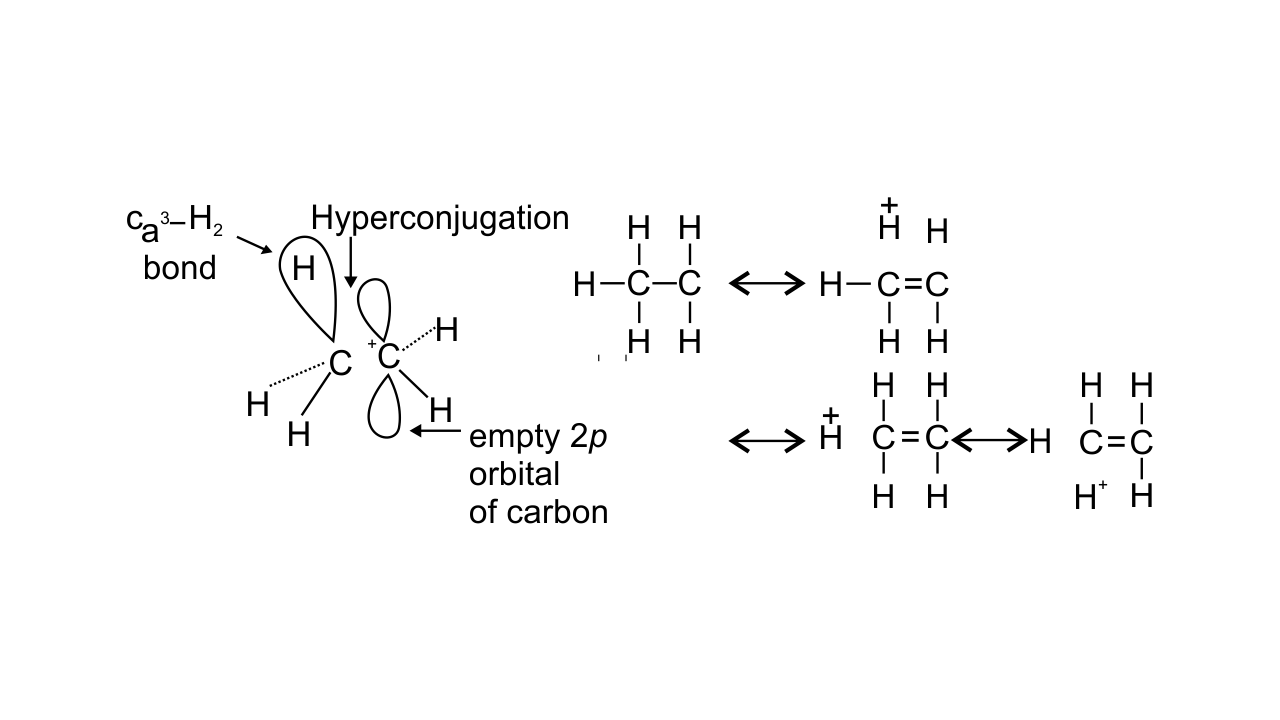Note : Consider structures I to VII and answer the questions 23–26.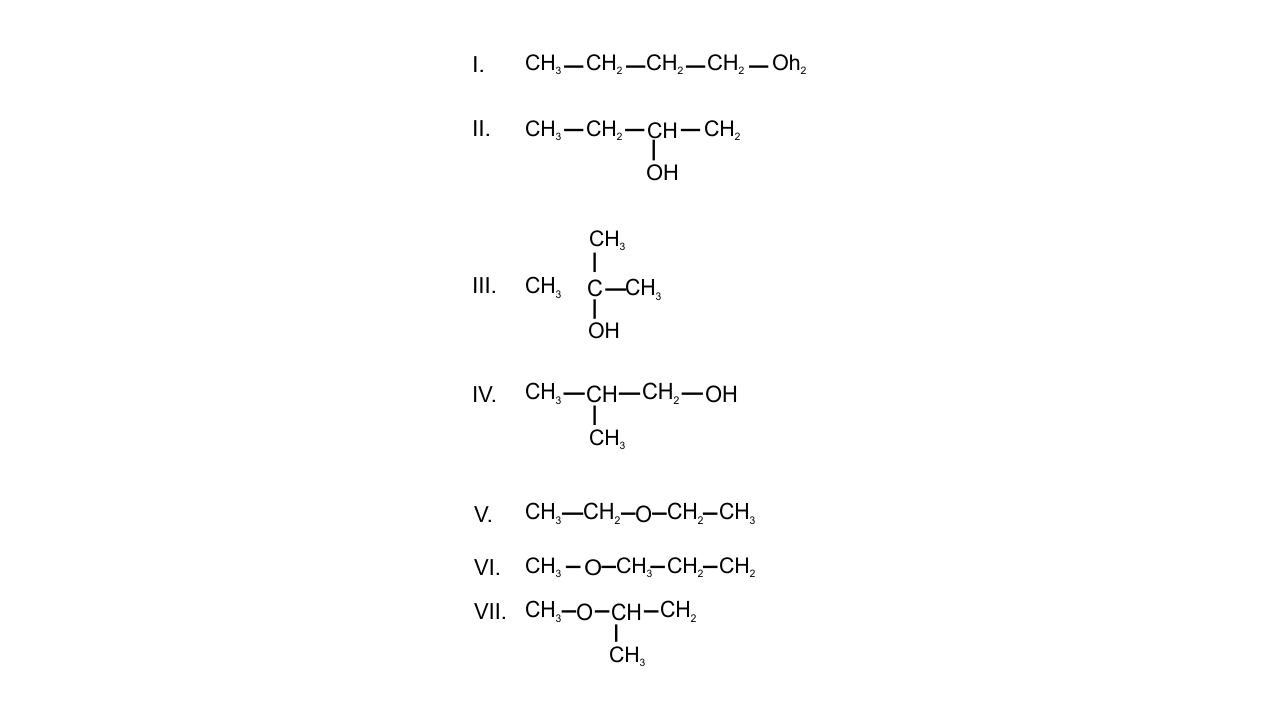23. Which of the above compounds form pairs of metamers?

Ans: If two or more compounds have the same molecular formula but different alkyl groups on either side of the functional groups then the compounds are said to be metamers.

In the given structures-

V and VI and VI and VII form a pair of metamers because alkyl groups are different on either side of the functional groups.

24. Identify the pairs of compounds which are functional group isomers.

Ans: The compounds with the same molecular formula but different functional groups are said to be functional group isomers.

Alcohols are found to be the functional isomers of ether. In the given structures, I, II, III, IV  alcohol functional group is present and V, VI, VII contains ether functional group. Hence, I and V, I and VI, I and VII, II and V, II and VI, II and VII, III and V, III and VI etc are functional group isomers.

25. Identify the pairs of compounds that represents position isomerism.

Ans: If two or more compounds differ in the position of substituent, functional group, or multiple bonds but molecular formula is same then these are said to be position isomers as they exhibit position isomerism.

In the given structures, I and II, III and IV, VI and VII are found to be the position isomers.

26. Identify the pairs of compounds that represents chain isomerism.

Ans: If two or more compounds have the same molecular formula but different carbon skeletons then these compounds are referred to as chain isomers and the phenomenon is termed as chain isomerism. The pairs of compounds that represent chain isomerism are- I, II, III, IV.

27. For testing halogens in an organic compound with $\mathbf{AgN}{{\mathbf{O}}_{\mathbf{3}}}$ solution, sodium extract (Lassaigne’s test) is acidified with dilute $\mathbf{HN}{{\mathbf{O}}_{\mathbf{3}}}$. What will happen if a student acidifies the extract with dilute ${{\mathbf{H}}_{\mathbf{2}}}\mathbf{S}{{\mathbf{O}}_{\mathbf{4}}}$ in place of dilute $\mathbf{HN}{{\mathbf{O}}_{\mathbf{3}}}$?

Ans: Elements such as nitrogen, halogens, Sulphur, and phosphorus present in an organic compound converted into ions by fusing with sodium metal followed by plucking in distilled water getting sodium extract (Lassaigne’s test). On adding dil. ${{\text{H}}_{\text{2}}}\text{S}{{\text{O}}_{\text{4}}}$ in place of dil. $\text{HN}{{\text{O}}_{\text{3}}}$for testing halogens by $\text{AgN}{{\text{O}}_{\text{3}}}$ , white precipitate of AgSO4 is formed. This will lead wrong result of chloride. Hence, only $\text{HN}{{\text{O}}_{\text{3}}}$is used instead of dil. ${{\text{H}}_{\text{2}}}\text{S}{{\text{O}}_{\text{4}}}$

28. What is the hybridisation of each carbon in ${{\mathbf{H}}_{\mathbf{2}}}\mathbf{C}\text{ }=\text{ }\mathbf{C}\text{ }=\text{ }\mathbf{C}{{\mathbf{H}}_{\mathbf{2}}}$.

Ans: The given structure is of ${{\text{C}}_{\text{3}}}{{\text{H}}_{\text{4}}}$ in which all three carbon atoms are linked to each other by double bonds. Carbons are marked as ${{\text{C}}_{\text{l}}}\text{, }{{\text{C}}_{\text{2}}}$ and ${{\text{C}}_{\text{3}}}$. ${{\text{C}}_{\text{l}}}$ and ${{\text{C}}_{\text{3}}}$ are $\text{s}{{\text{p}}^{\text{2}}}$hybridized as containing 3σ bonds and 1π bond. ${{\text{C}}_{\text{2}}}$ has 2 σ bonds and 2 π bonds so, it is sp hybridized.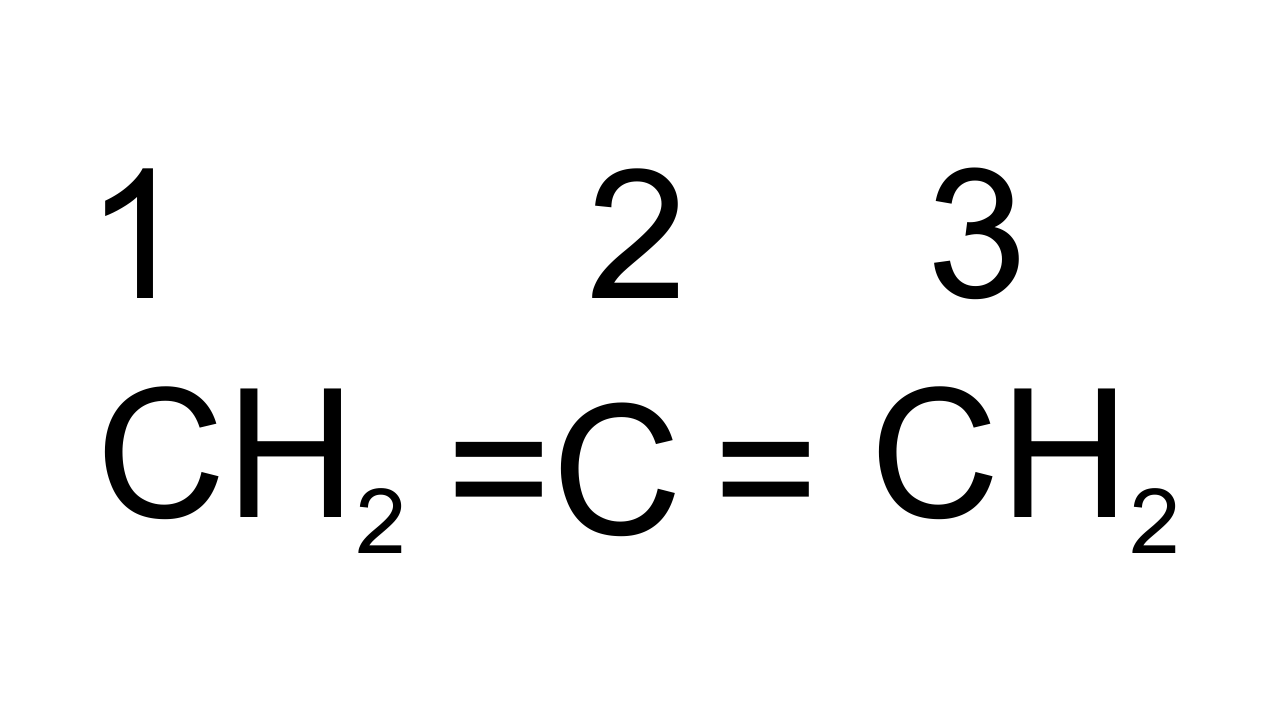29. Explain, how is the electronegativity of carbon atoms related to their state of hybridisation in an organic compound?

Ans: Electronegativity of an element is directly proportional to its  s-character. If C is $\text{s}{{\text{p}}^{3}}$hybridized then s- character is 25%, if it is $\text{s}{{\text{p}}^{\text{2}}}$ hybridized then s-character is 33% and if sp hybridized then s-character is 50%. Hence, sp hybridized carbon has strong hybridization, s electrons are more strongly attracted by nucleus than p-electrons thus electronegativity of carbon increases with increase in s-character.

30. Show the polarisation of carbon-magnesium bond in the following structure.

$\mathbf{C}{{\mathbf{H}}_{\mathbf{3}}}\mathbf{C}{{\mathbf{H}}_{\mathbf{2}}}\mathbf{C}{{\mathbf{H}}_{\mathbf{2}}}\mathbf{C}{{\mathbf{H}}_{\mathbf{2}}}\mathbf{Mg}\mathbf{X}$

Ans: Carbon is known to be more electronegative than Magnesium and hence Mg acquires partial positive charge while C acquires partial negative charge because the bonded pair of electrons is attracted towards it.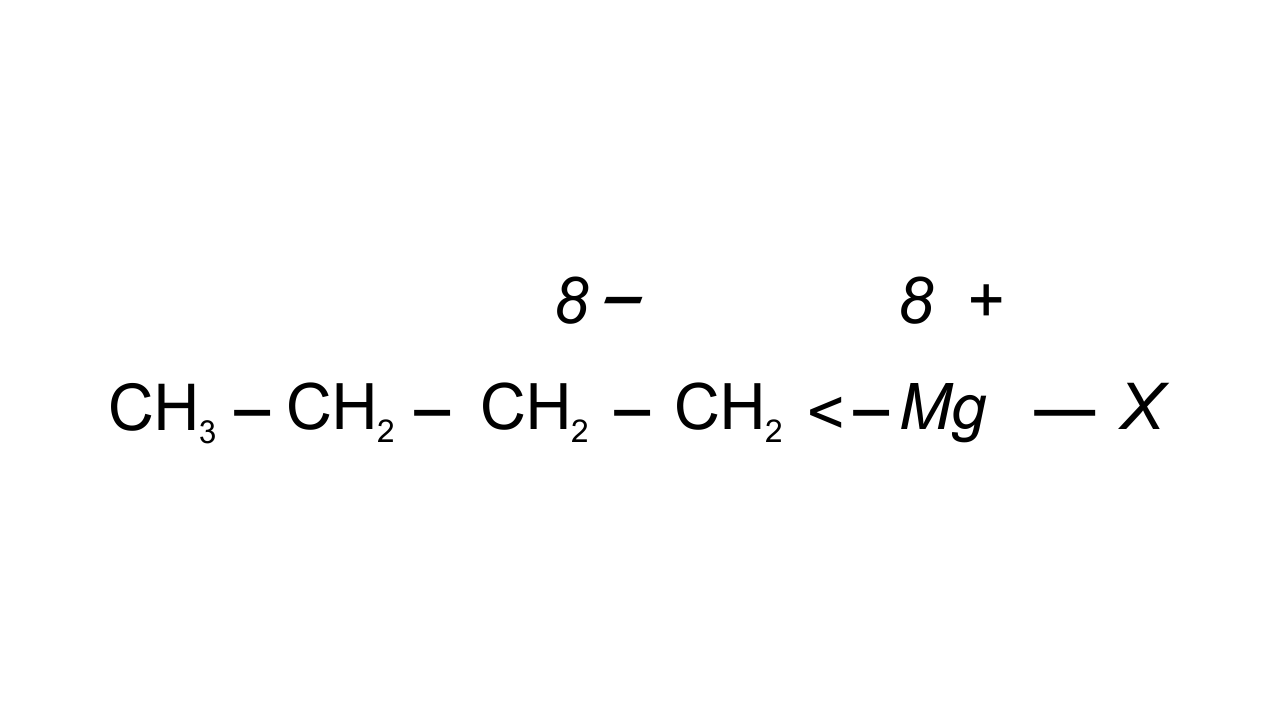31. Compounds with same molecular formula but differing in their structures are said to be structural isomers. What type of structural isomerism is shown byAns: These are position isomers as if two or more compounds differ in the position of substituents, functional groups, or multiple bonds but if the molecular formula is same then these are said to be position isomers. Here the functional groups are attached to different carbon atoms in the carbon chain, but the molecular formula is the same. They are not metamers because the number of C atoms on either side of-S- are the same.

32. Which of the following selected chains is correct to name the given compound according to IUPAC system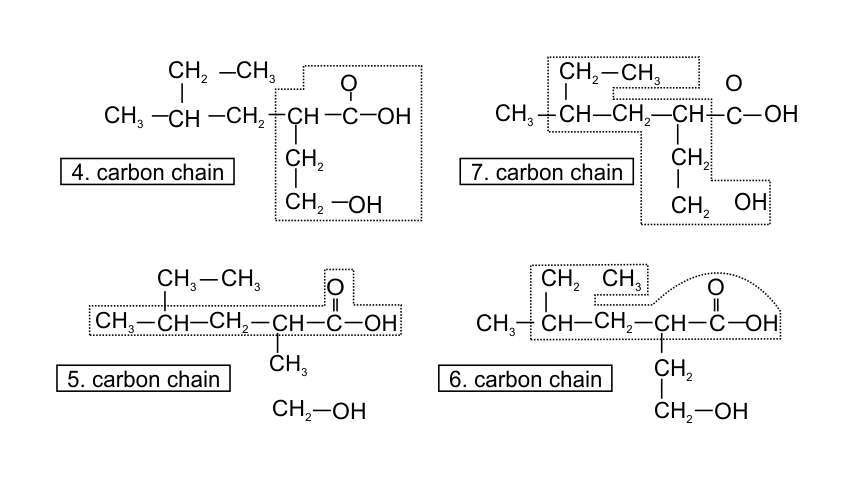Ans: In accordance with the IUPAC nomenclature, the longest carbon chain is selected such that it must have maximum functional groups present in the compound. Therefore, only in one selected chain of 4 carbon atoms including both the functional group is corrected one.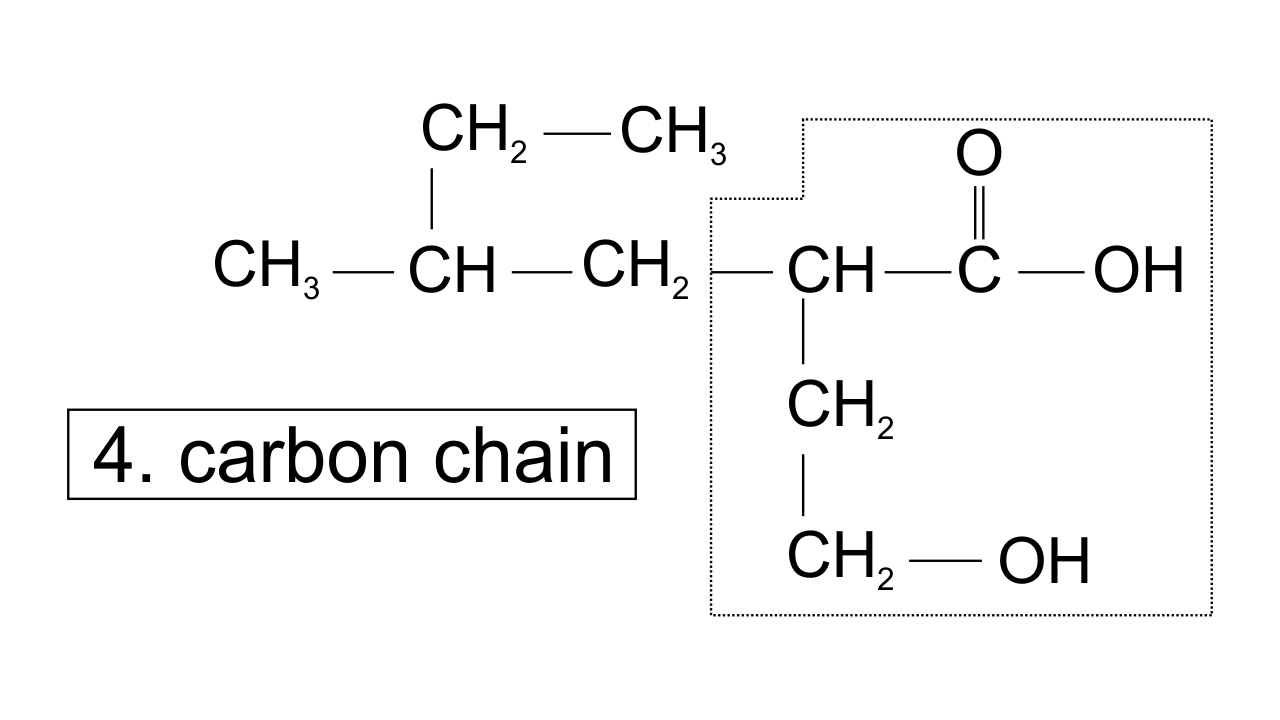While in the other three, carbon atoms are in the selected chain, but both the functional groups are not included.

33. In DNA and RNA, nitrogen atom is present in the ring system. Can Kjeldahl method be used for the estimation of nitrogen present in these? Give reasons.

Ans:  In DNA and RNA , nitrogen is present in heterocyclic base and also present in the ring but not as a substituent. Therefore, nitrogen present in the ring cannot be converted into${{\left( \text{N}{{\text{H}}_{\text{4 }}} \right)}_{\text{2}}}\text{S}{{\text{O}}_{\text{4}}}$. Hence, it cannot be estimated by Kjeldahl method.

34. If a liquid compound decomposes at its boiling point, which method (s) can you choose for its purification. It is known that the compound is stable at low pressure, steam volatile and insoluble in water.

Ans:  If a liquid compound decomposes at its boiling point but is steam volatile and insoluble in water and stable at low pressure. Then steam distillation method can be used for its purification.

Note : Answer the questions 35 to 38 on the basis of information given below:

“Stability of carbocations depends upon the electron releasing inductive effect of groups adjacent to positively charged carbon atom involvement of neighbouring groups in hyperconjugation and resonance.”

35. Draw the possible resonance structures for $\mathbf{C}{{\mathbf{H}}_{\mathbf{3}}}-\underset{\mathbf{\bullet \bullet }}{\overset{\mathbf{\bullet \bullet }}{\mathop{\mathbf{O}}}}\,-\mathbf{C}{{\mathbf{H}}_{\mathbf{2}}}^{\mathbf{+}}$ and predict which of the structures is more stable. Give reason for your answer.

Ans: The resonating structures can be drawn as-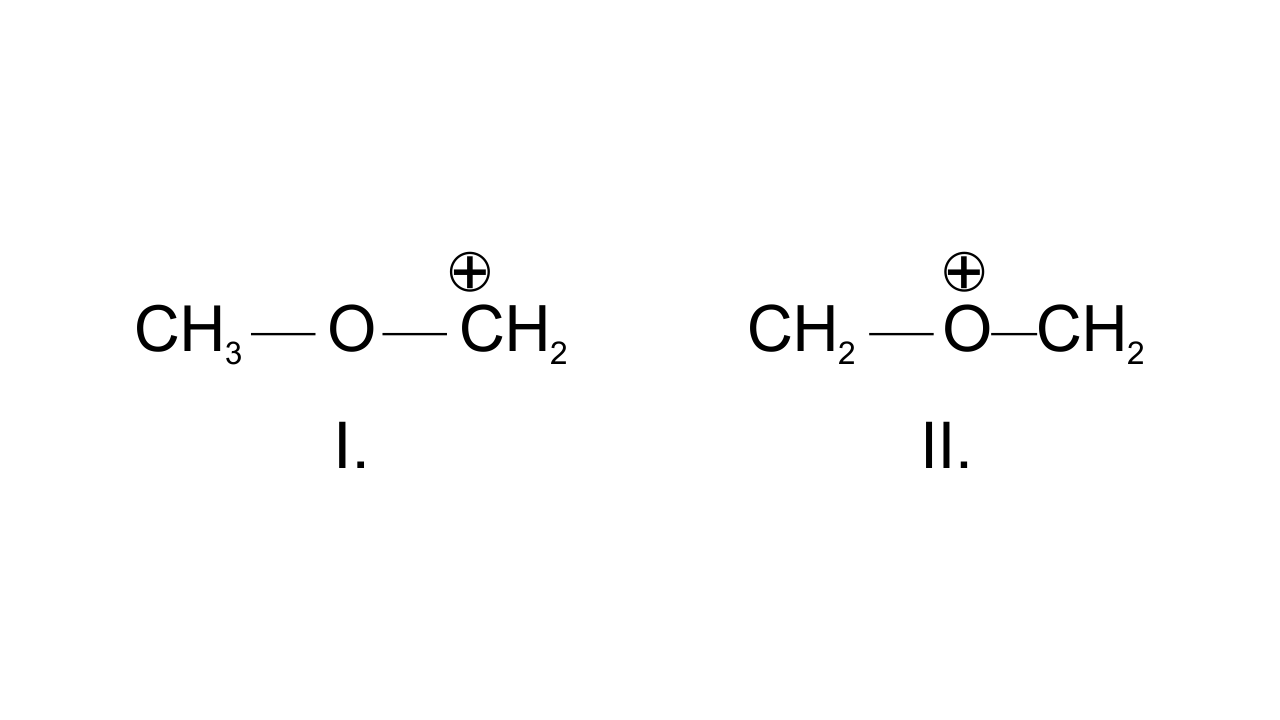In structure I, $\text{C}{{\text{H}}_{\text{2}}}$group has the positive charge which means octet is not completed, but in structure II, both the carbon atoms and oxygen atom have complete octet hence, more stable.

36. Which of the following ions is more stable? Use resonance to explain your answer.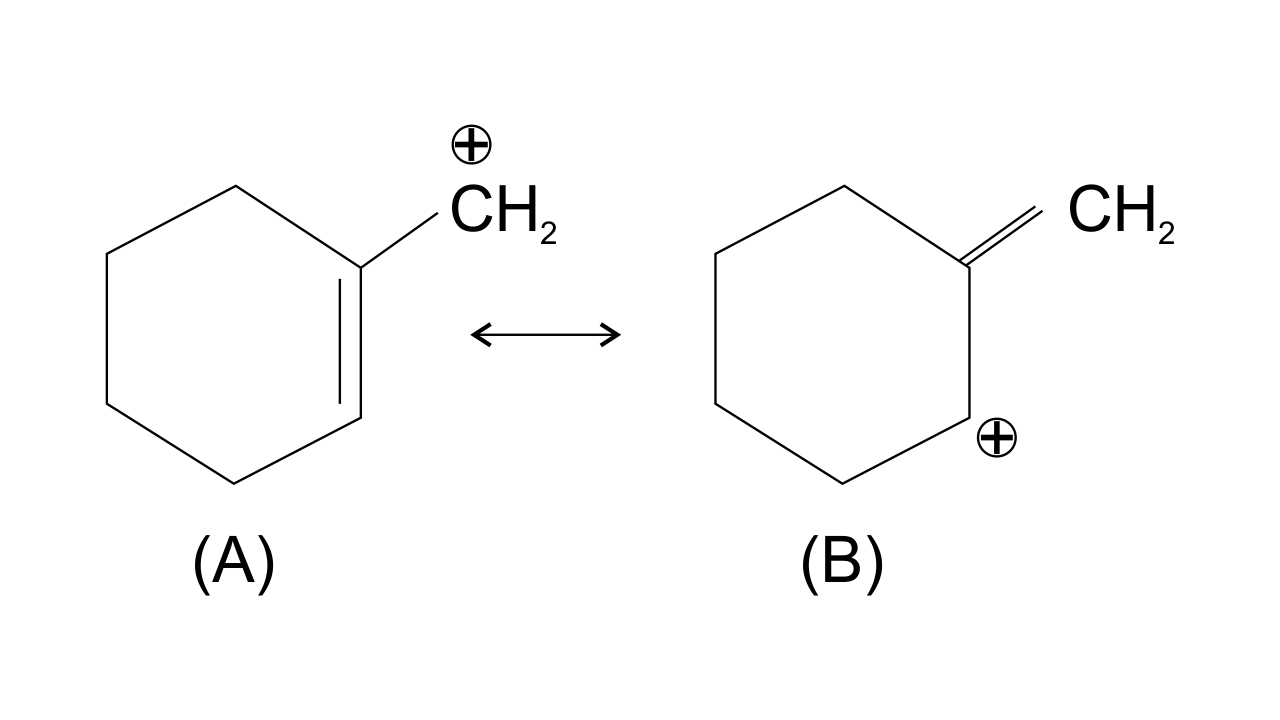Ans: The structure A is more stable than structure B. This is because carbocation A is more Planar and π electrons from the ring shift to the side group and are stabilized by resonance while structure B is non-planar and does not undergo resonance. Also, double bond is more stable within the ring as compared to the side chain.

37. The structure of triphenylmethyl cation is given below. This is very stable and some of its salts can be stored for months. Explain the cause of high stability of this cation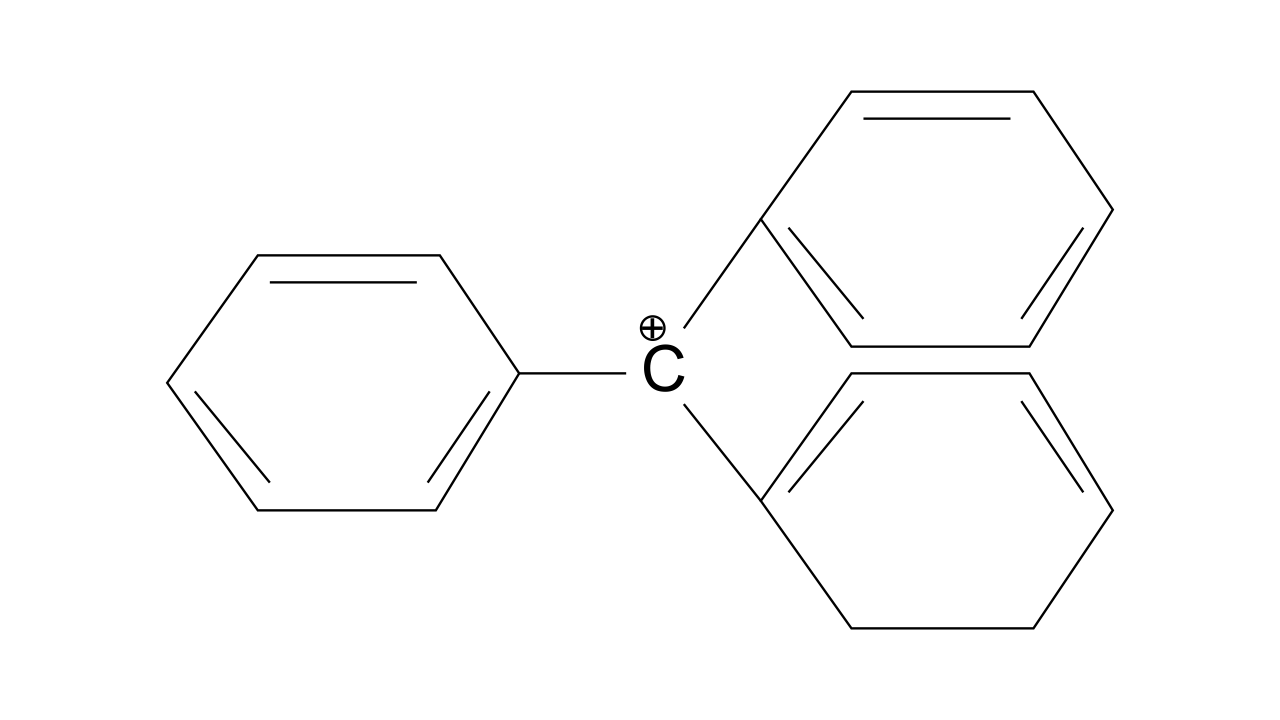Ans: Triphenylmethyl cation is found to be very much stable as this positive charge on methyl carbon is delocalized in three phenyl rings. In each phenyl ring, positive charge is developed on 2 ortho position and para position, i.e., three resonating structures. Total resonating structures given by triphenylmethyl cation are nine. Hence, it is very stable. These structures can be shown as.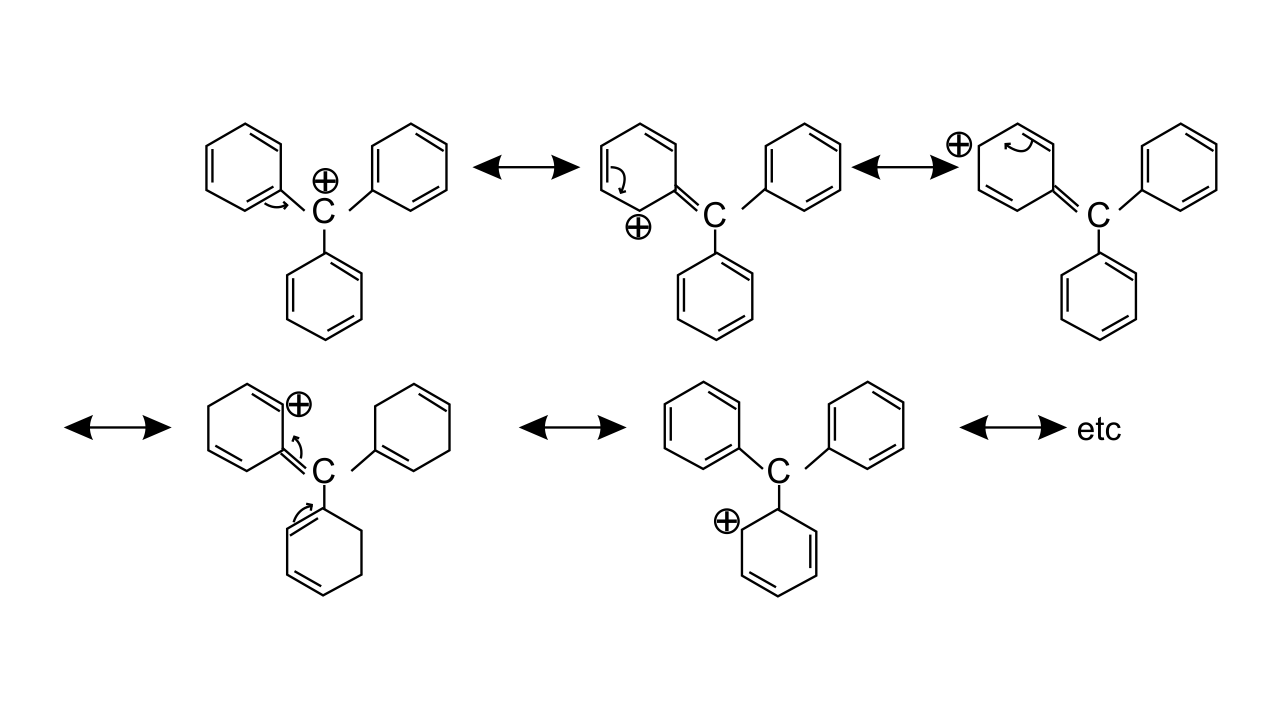38. Write structures of various carbocation’s that can be obtained from 2- methyl butane. Arrange these carbocation’s in order of increasing stability.

Ans: Four possible carbocations can be obtained from 2- methyl butane  are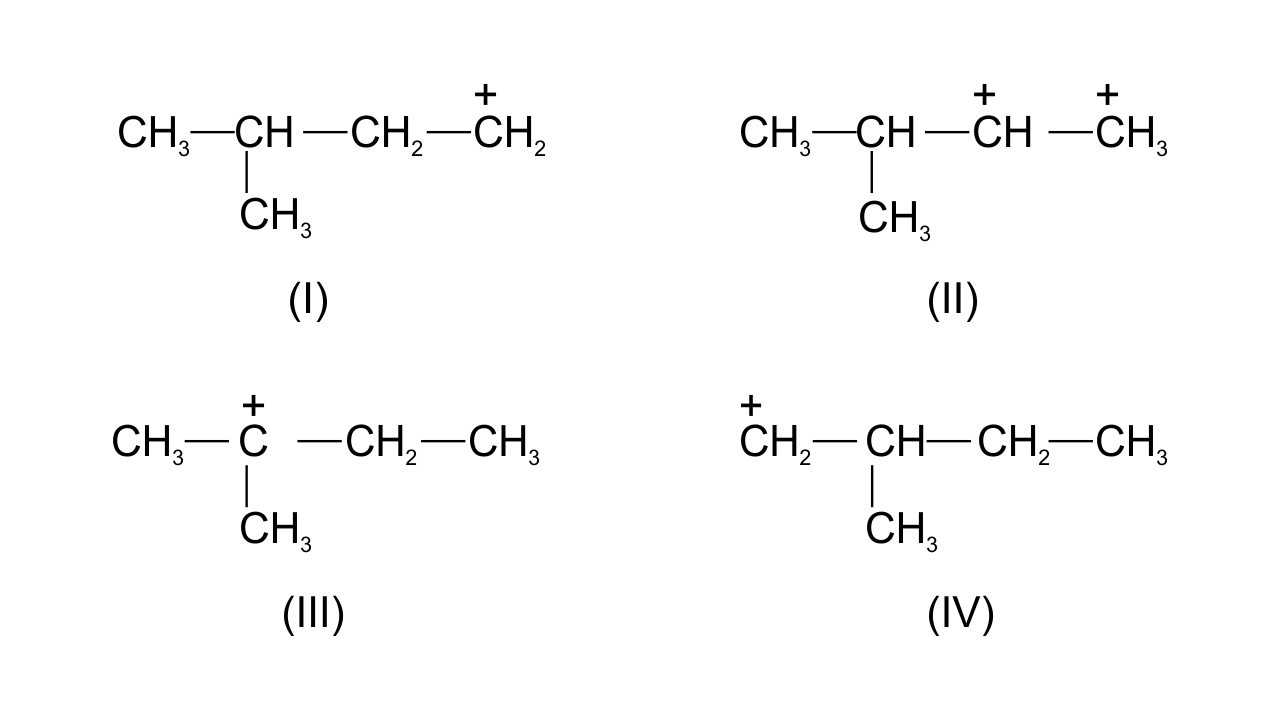Generally, order of stability follows the order-

1° carbocation < 2° carbocation < 3° carbocation

Order of increasing stability of the given structures is-

I < IV < II < III.

39. Three students, Manish, Ramesh and Rajni were determining the extra elements present in an organic compound given by their teacher. They prepared the Lassaigne’s extract (L.E.) independently by the fusion of the compound with sodium metal. Then they added solid $\mathbf{FeS}{{\mathbf{O}}_{\mathbf{4}}}$and dilute Sulphur acid to a part of Lassaigne’s extract. Manish and Rajni obtained Prussian blue colour, but Ramesh got red colour. Ramesh repeated the test with the same Lassaigne’s extract, but again got red colour only. They were surprised and went to their teacher and told him about their observation. Teacher asked them to think over the reason for this. Can you help them by giving the reason for this observation? Also, write the chemical equations to explain the formation of compounds of different colours.

Ans: Whenever an organic compound contains both N and S, then on fusion with Na metal compound gives NaSCN or NaCN and $\text{N}{{\text{a}}_{\text{2}}}\text{S}$ depending on the quantity of Na metal. If  Na metal is less, then only NaSCN is formed. In that case L.E on treating with $\text{FeS}{{\text{O}}_{\text{4}}}$ and ${{\text{H}}_{\text{2}}}\text{S}{{\text{O}}_{\text{4}}}$ gives red colour due to the formation of ferric thiocyanide $\text{Fe}{{\left( \text{SCN} \right)}_{\text{3}}}$. In case of NaCN, L.E on treating with $\text{FeS}{{\text{O}}_{\text{4}}}$ and ${{\text{H}}_{\text{2}}}\text{S}{{\text{O}}_{\text{4}}}$gives Prussian blue colour.

Chemical reactions: $\text{F}{{\text{e}}^{\text{2+ }}}\to \text{F}{{\text{e}}^{\text{3+}}}$ (Oxidation)

$\text{F}{{\text{e}}^{\text{3+}}}\text{ + 3NaSCN }\to \text{Fe}{{\left( \text{SCN} \right)}_{\text{3}}}\text{ + 3N}{{\text{a}}^{\text{+}}}\text{ }$

Red colour

If Na is in excess then :

$\text{NaSCN + 2Na }\xrightarrow{\Delta }\text{NaCN + N}{{\text{a}}_{\text{2}}}\text{S }$

\begin{align} & \text{6C}{{\text{N}}^{\text{-}}}\text{ + F}{{\text{e}}^{\text{2+}}}\text{ }\to \text{ }\left[ \text{Fe}\left( \text{CN} \right){{\text{ }}_{\text{6}}} \right]{{\text{ }}^{\text{4-}}}\text{ } \\ & \text{3}{{\left[ \text{Fe}{{\left( \text{CN} \right)}_{\text{6}}}\text{ } \right]}^{\text{4-}}}\text{ + 4F}{{\text{e}}^{\text{3+ }}}\xrightarrow{\text{x}{{\text{H}}_{\text{2}}}\text{O}}\text{F}{{\text{e}}_{\text{4}}}\text{ }{{\left[ \text{Fe}{{\left( \text{CN} \right)}_{\text{6 }}} \right]}_{\text{3}}}\text{ }\text{.x}{{\text{H}}_{\text{2}}}\text{O} \\ \end{align}

Prussian blue

40. Name the compounds whose line formulae are given below :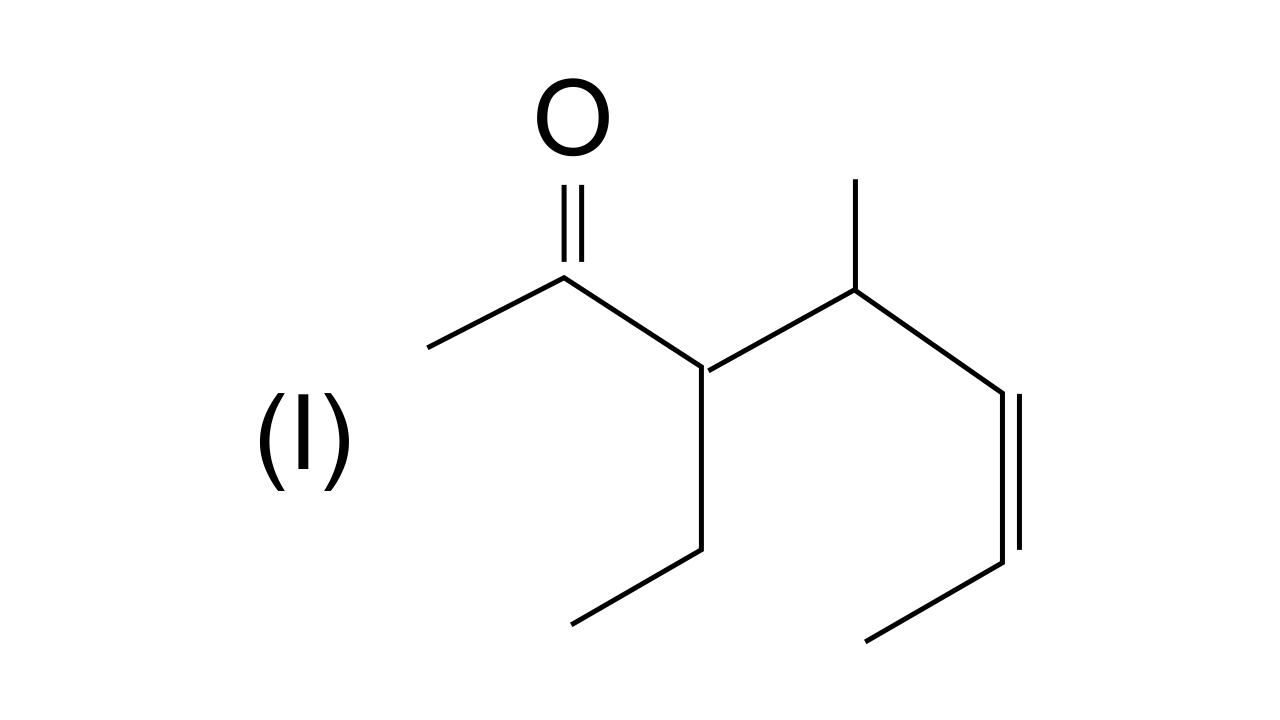Ans: In this structure i.e., 3-ethyl, 4-methylhept-5-en-2-one. (Longest carbon  chain is selected in such a way that the functional group > C = O gets the lowest possible locant.

Ans: In this structure  i.e.,  3-nitrocyclohex-l-en (Carbon atoms of the ring are numbered in such a way that double bonded carbon gets the lowest number followed by the nitro group $\text{-N}{{\text{O}}_{\text{2}}}$.

41. Write structural formulae for compounds named as-

(a) 1-Bromoheptane

Ans: $\text{C}{{\text{H}}_{\text{3 }}}\text{-C}{{\text{H}}_{\text{2}}}\text{ -C}{{\text{H}}_{\text{2}}}\text{ -C}{{\text{H}}_{\text{2}}}\text{ -C}{{\text{H}}_{\text{2}}}\text{ -C}{{\text{H}}_{\text{2}}}\text{ -C}{{\text{H}}_{\text{2}}}\text{Br}$

l-Bromoheptane

(b) 5-Bromoheptanoic acid

Ans: $\text{C}{{\text{H}}_{\text{3}}}\text{ -C}{{\text{H}}_{\text{2}}}\text{ -CH}\left( \text{Br} \right)\text{-C}{{\text{H}}_{\text{2}}}\text{ -C}{{\text{H}}_{\text{2}}}\text{ -C}{{\text{H}}_{\text{2}}}\text{ -COOH}$

5-Bromoheptanoic acid

42. Draw the resonance structures of the following compounds.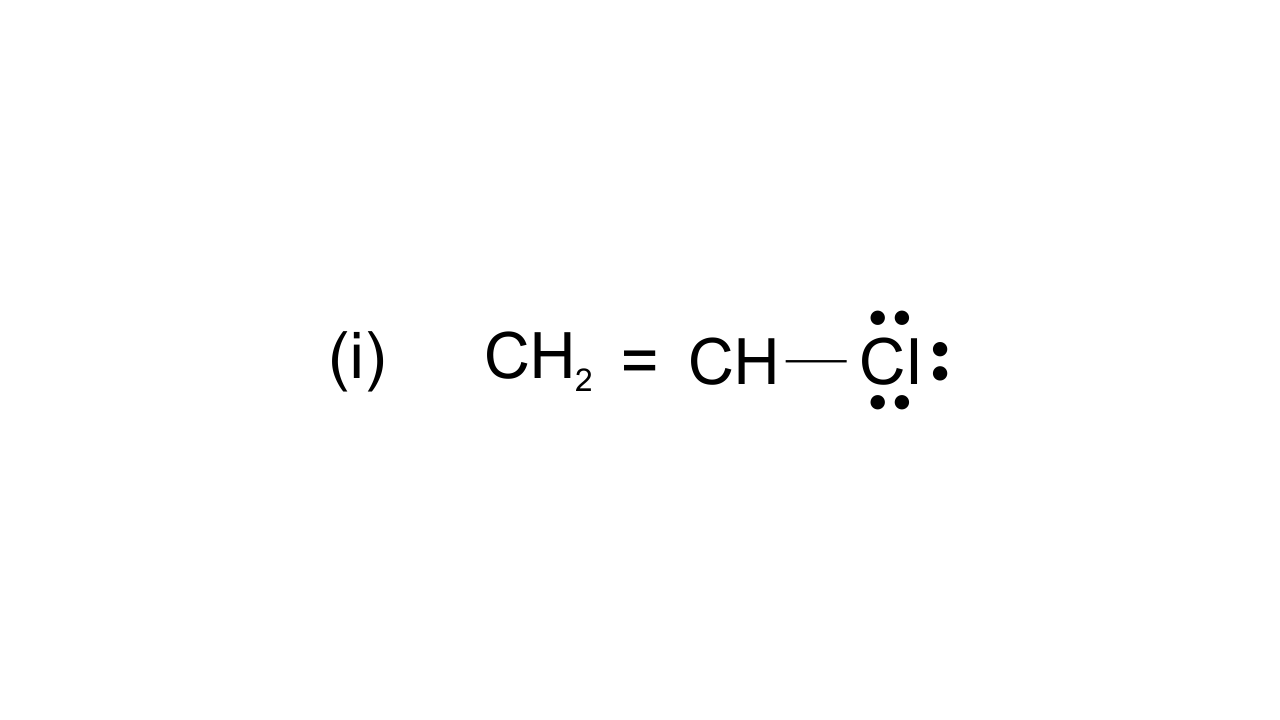Ans: $\text{ }\!\!~\!\!\text{ C}{{\text{H}}_{\text{2}}}\text{=CH-CI}\leftrightarrow \text{C}{{\text{H}}_{\text{2}}}\text{-CH=C}{{\text{I}}^{\text{+}}}$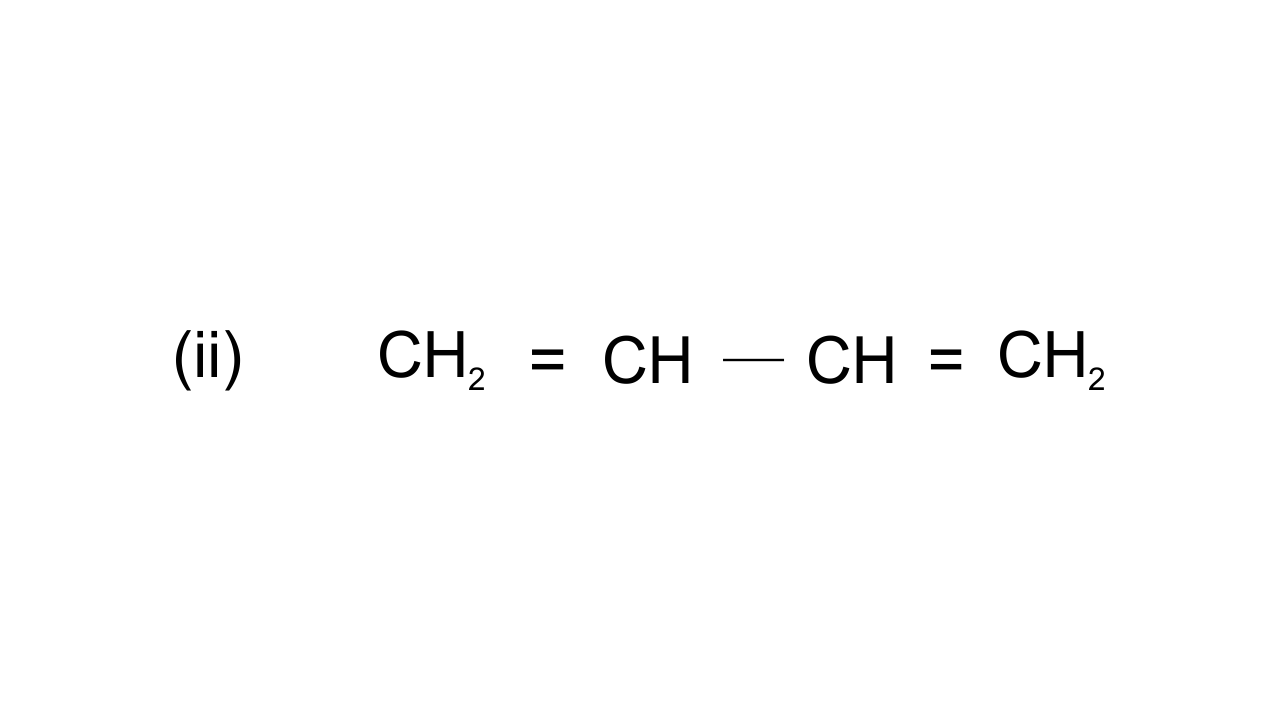Ans: $\text{C}{{\text{H}}_{\text{2}}}\text{=CH-CH=C}{{\text{H}}_{\text{2}}}\leftrightarrow \text{C}{{\text{H}}_{\text{2}}}\text{-CH=CH=C}{{\text{H}}_{\text{2}}}\leftrightarrow \text{CH=CH-C}{{\text{H}}_{\text{2}}}^{\text{+}}$

$\left( \text{iii} \right)\text{C}{{\text{H}}_{\text{2}}}\text{=CH-}\underset{\begin{smallmatrix} \,\text{ }\!\!|\!\!\text{ } \\ \text{H} \end{smallmatrix}}{\mathop{\text{C}}}\,\text{=O}$

Ans: $\text{C}{{\text{H}}_{\text{2}}}\text{=CH-C}\text{HO}\leftrightarrow \overset{\text{+}}{\mathop{\text{C}{{\text{H}}_{\text{2}}}}}\,\text{-CH=CH}{{\text{O}}^{\text{-}}}$

43. Identify the most stable species in the following set of ions giving reasons :

$\overset{\text{+}}{\mathop{\text{C}}}\,{{\text{H}}_{\text{3}}}\text{,}\overset{\text{+}}{\mathop{\text{C}}}\,{{\text{H}}_{\text{2}}}\text{Br,}\overset{\text{+}}{\mathop{\text{C}}}\,\text{HB}{{\text{r}}_{\text{2}}}\text{,}\overset{\text{+}}{\mathop{\text{C}}}\,\text{B}{{\text{r}}_{\text{2}}}$

Ans: In this case $\text{C}{{\text{H}}_{\text{3}}}^{\text{+}}$ is the most stable species because replacement of H atom by Br atom (-Inductive effect) increases positive charge on the carbon atom and destabilizes the species.

(ii) $\overset{\oplus }{\mathop{\text{C}}}\,{{\text{H}}_{3}}$$\overset{\oplus }{\mathop{\text{C}}}\,{{\text{H}}_{2}} \text{Cl}, \overset{\oplus }{\mathop{\text{C}}}\,\text{HC}{{\text{l}}_{2}} \overset{\oplus }{\mathop{\text{C}}}\,$$\text{C}{{\text{l}}_{3}}$

Ans: $\overset{\oplus }{\mathop{\text{C}}}\,\text{C}{{\text{l}}_{3}}$is most stable because negative charge on carbon atom is dispersed due to -I effect of Cl. More Cl atoms, more dispersal of negative charge and more stabilized.

44. Give three points of differences between inductive effect and resonance effect.

Ans:

 S.No. Inductive Effect Resonance Effect 1 It involves the displacement of σ electrons in saturated compounds. It involves displacement of $\text{ }\!\!\pi\!\!\text{ }$electrons or Lone pair of electrons in unsaturated and conjugated compounds. 2 In inductive effect, there is slightdisplacement of σ electrons andpartial positive or negative charge develops. In this effect, there is complete transfer of $\text{ }\!\!\pi\!\!\text{ }$ electrons and as a result, complete positive or negative charge develops. 3 Inductive effect can move only up to 3 to 4 carbons. In  this case, movement of electrons along the length a conjugated system takes place.

45. Which of the following compounds will not exist as resonance hybrid.   Give reason for your answer:

$\left( \mathbf{i} \right)\mathbf{ C}{{\mathbf{H}}_{\mathbf{3}}}\mathbf{OH~}$

Ans: $\text{C}{{\text{H}}_{\text{3}}}\text{OH}$ -It does not show resonance due to the absence of π electrons.

$\left( \mathbf{ii} \right)\mathbf{ R-CON}{{\mathbf{H}}_{\mathbf{2 }}}$

Ans: It shows resonance due to the presence of lone pairs on the Nitrogen atom and π electrons on the C = O bond. Hence, amide can be represented by three resonating structures.

$\left( \mathbf{iii} \right)\mathbf{ C}{{\mathbf{H}}_{\mathbf{3}}}\mathbf{CH = CHC}{{\mathbf{H}}_{\mathbf{2}}}\mathbf{N}{{\mathbf{H}}_{\mathbf{2}}}$

Ans: $\text{C}{{\text{H}}_{\text{3}}}\text{CH = CHC}{{\text{H}}_{\text{2}}}\text{N}{{\text{H}}_{\text{2}}}$- In this structure, the lone pair of electrons present on Nitrogen atom, is not conjugated with the π electrons of double bond. Hence, it does not show resonance.

46. Why does $\mathbf{S}{{\mathbf{O}}_{\mathbf{3}}}$act as an electrophile?

Ans: $\text{S}{{\text{O}}_{\text{3}}}$ acts as an electrophile as in this, three highly electronegative oxygen atoms are attached to the sulphur atom which makes it electron deficient. It can be shown by resonance that it gets positive charge and acts as an electrophile.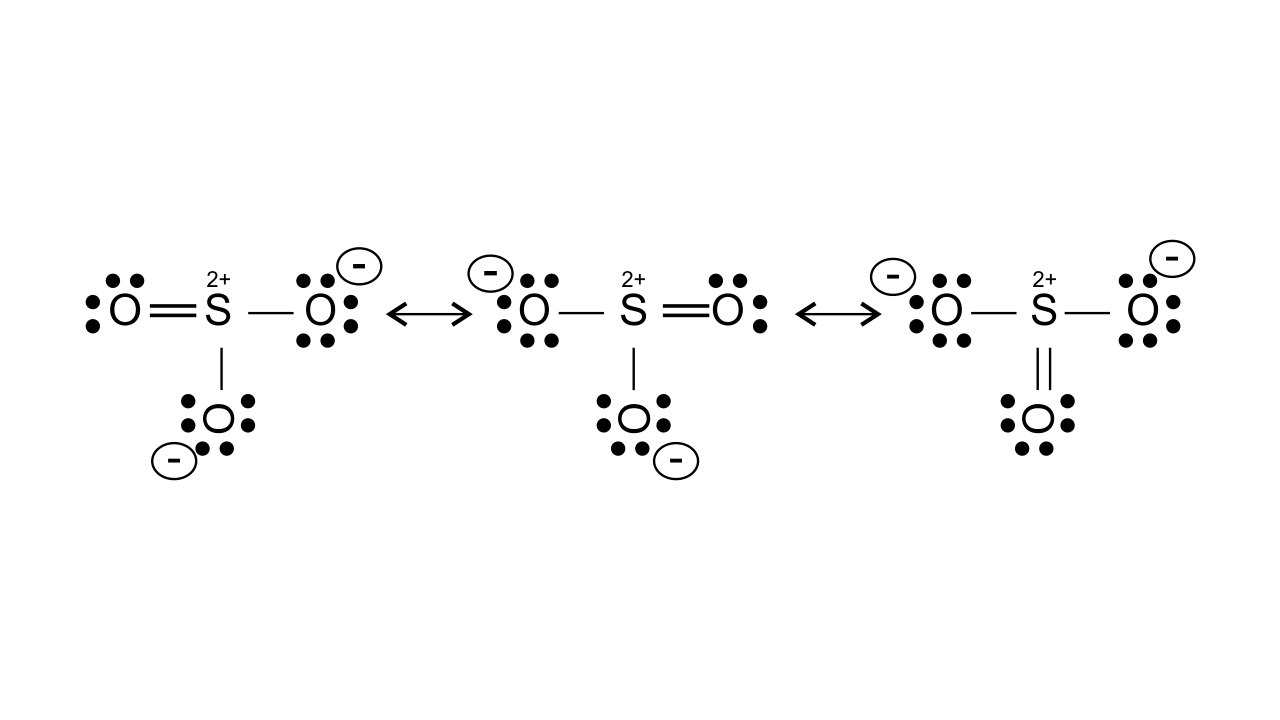47. Resonance structures of propenal are given below. Which of these resonating structures is more stable? Give reason for your answer.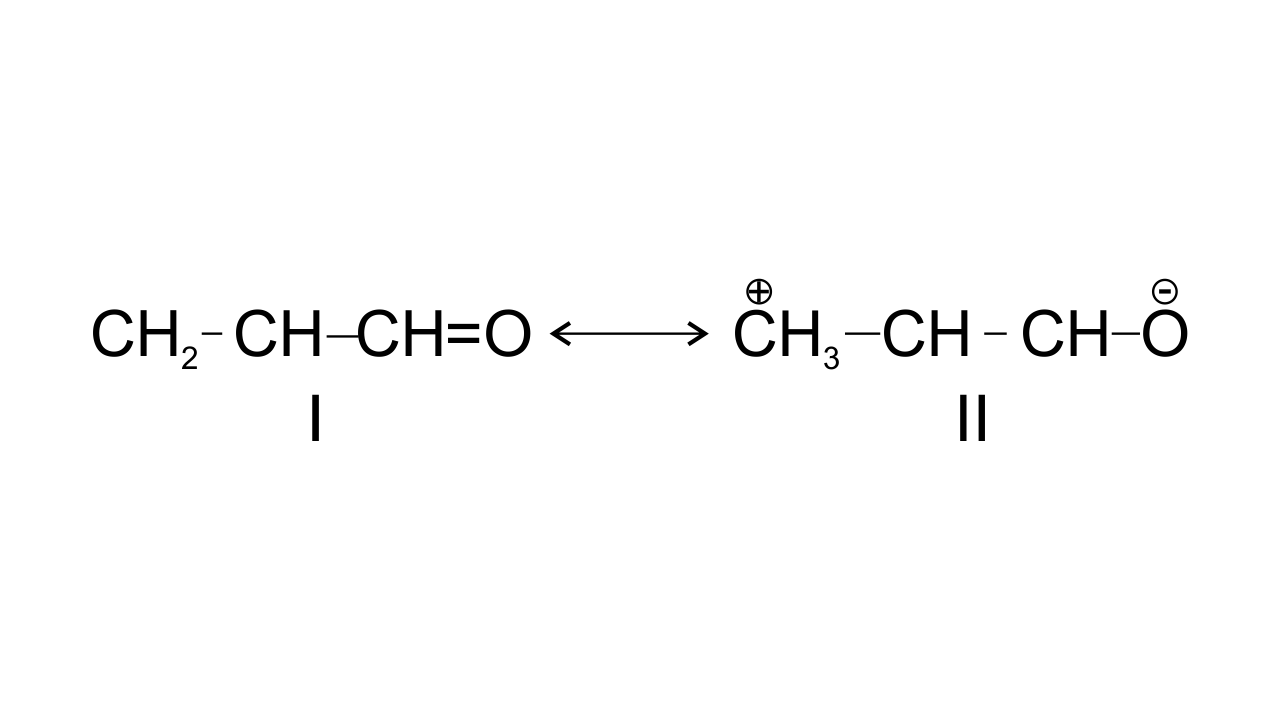Ans:

The structure-I of propenal is found to be more stable because structure having more covalent bonds in a resonating structure is found to have more stability, in structure II, the terminal carbon has only six electrons which makes it less stable.

$\text{C}{{\text{H}}_{\text{2}}}\text{=CH---CH=O }$    $\leftrightarrow$     $\text{ }\overset{+}{\mathop{\text{C}}}\,{{\text{H}}_{\text{2}}}\text{---CH=CH---}\overset{\text{-}}{\mathop{\text{O}}}\,\text{ }$

Structure-I                                 Structure-II

48. By mistake, an alcohol (boiling point 97°C) was mixed with a hydrocarbon (boiling point 68°C). Suggest a suitable method to separate the two compounds. Explain the reason for your choice.

Ans: Mixture of alcohol and hydrocarbon can be separated by simple distillation because both the components have large differences in their boiling points. When temperature is raised to the low boiling liquid i.e., hydrocarbon, the vapours consist only of hydrocarbons without any contamination of alcohol.

49. Which of the two structures (A) and (B) given below is more stabilised by resonance? Explain.

$\mathbf{C}{{\mathbf{H}}_{\mathbf{3}}}\mathbf{COOH}$               and                       $\mathbf{C}{{\mathbf{H}}_{\mathbf{3}}}\mathbf{CO}{{\mathbf{O}}^{-}}$

A                                                   B

Ans: Resonating structure of $\text{C}{{\text{H}}_{\text{3}}}\text{COOH}$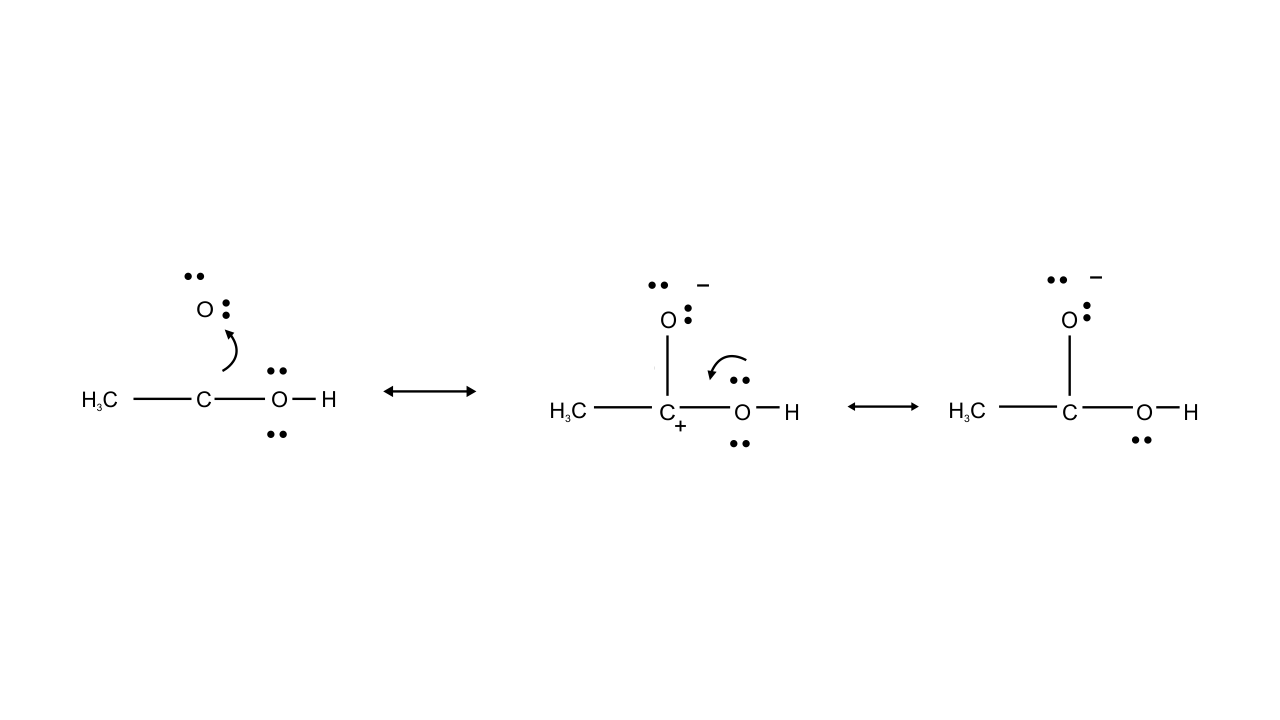In this case structure II is less stable than I because in structure II separation of positive and negative charges is there. Therefore, contribution of structure (II) is less than that of (I), these hybrids of compound (A) i.e., $\text{C}{{\text{H}}_{\text{3}}}\text{COOH}$.

Resonating structure of $\text{C}{{\text{H}}_{\text{3}}}\text{CO}{{\text{O}}^{\text{-}}}$-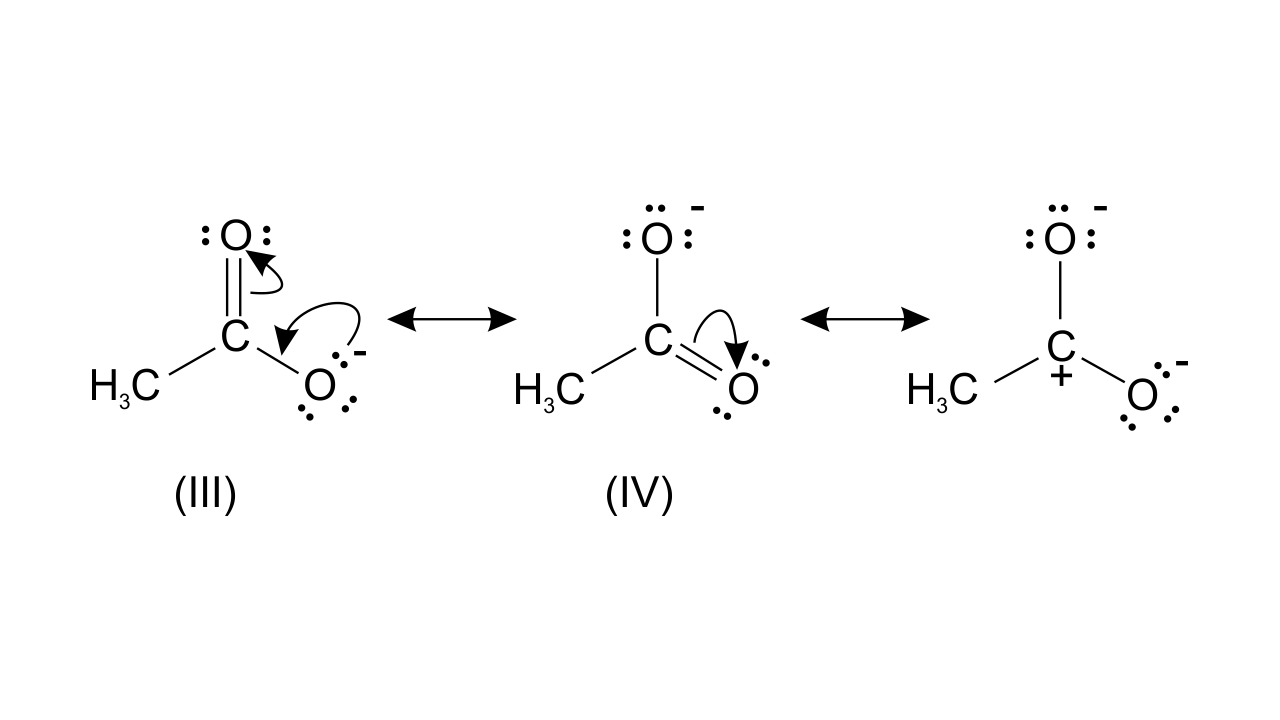While structure (III) and (IV) are of equal energy and also contribute equally towards, resonance hybrid of compound (B). Therefore, $\text{C}{{\text{H}}_{\text{3}}}\text{CO}{{\text{O}}^{\text{-}}}$ is more stable.

### Matching Type

In the following questions more than one correlation is possible between options of Column I and Column II. Make as many correlations as you can.

50. Match the type of mixture of compounds in Column I with the technique of separation/purification given in Column II.

 Column I Column II (i) Two solids which have different solubility’s in a solvent, and which do not undergo reaction when dissolved in it. (a) Steam distillation (ii) Liquid that decomposes at its boiling point (b) Fractional distillation (iii) Steam volatile liquid (c) Simple distillation (iv) Two liquids which have boiling points close to each other (d)Distillation under reduced pressure (v) Two liquids with large difference in boiling points. (e) Crystallization

Ans:

 Column I Column II (i) Two solids which have different solubilities in a solvent, and which do not undergo reaction when dissolved in it. (e) Crystallization (ii) Liquid that decomposes at its boiling point (d)Distillation under      reduced pressure (iii) Steam volatile liquid (a) Steam distillation (iv) Two liquids which have boiling points close to each other (b) Fractional distillation (v) Two liquids with large differences in boiling points. (c) Simple distillation

51. Match the terms mentioned in Column I with the terms in Column II.

 Column I Column II (i) Carbocation (a) Cyclohexane and 1- hexane (ii) Nucleophile (b) Conjugation of electrons of C–H bond with empty p-orbital present at adjacent positively charged carbon. (iii) Hyperconjugation (c) $\mathbf{s}{{\mathbf{p}}^{\mathbf{2}}}$hybridized carbon with empty p-orbital (iv) Isomers (d) Ethyne (v) sp hybridization (e) Species that can receive a pair of electrons (vi) Electrophile (f) Species that can supply a pair of electrons

Ans:

 Column I Column II (i) Carbocation (c) $\text{s}{{\text{p}}^{\text{2}}}$hybridized carbon with empty p-orbital (ii) Nucleophile (f) Species that can supply a pair of electrons (iii) Hyperconjugation (b) Conjugation of electrons of C–H bond with empty p-orbital present at adjacent positively charged carbon. (iv) Isomers (a) Cyclohexane and 1- hexane (v) sp hybridization (d) Ethyne (vi) Electrophile (e) Species that can receive a pair of electrons

52. Match Column I with Column II.

 Column I Column II (i) Dumas method (a) $\mathbf{AgN}{{\mathbf{O}}_{\mathbf{3}}}$ (ii) Kjeldahl’s method (b) Silica gel (iii) Carius method (c) Nitrogen gas (iv) Chromatography (d) Free radicals (v) Hemolysis (e) Ammonium sulphate

Ans:

 Column I Column II (i) Dumas method (c) Nitrogen gas (ii) Kjeldahl’s method (e) Ammonium sulphate (iii) Carius method (a) $\text{AgN}{{\text{O}}_{\text{3}}}$ (iv) Chromatography (b) Silica gel (v) Hemolysis (d) Free radicals

53. Match the intermediates given in Column I with their probable structure in Column II.

Ans: (i) (a); (ii) (a); (iii) (b

 Column I Column II (i) Free radical (a) Trigonal planar (ii) Carbocation (b) Pyramidal (iii) Carbanion (c) Linear

Ans:

 Column I Column II (i) Free radical (a) Trigonal planar (ii) Carbocation (a) Trigonal planar (iii) Carbanion (b) Pyramidal

54. Match the ions given in Column I with their nature given in Column II.

 Column I Column II (i) $\text{C}{{\text{H}}_{3}}-\underset{\bullet \bullet }{\overset{\bullet \bullet }{\mathop{\text{O}}}}\,-\overset{\oplus }{\mathop{\text{C}}}\,\text{H-C}{{\text{H}}_{\text{3}}}$ (a) Stable due to resonance (ii) ${{\text{F}}_{3}}-\overset{\oplus }{\mathop{\text{C}}}\,$ (b) Destabilized due to inductive effect (Image will be uploaded soon) (c) Stabilized by hyperconjugation (vi) $\text{C}{{\text{H}}_{3}}-\overset{\oplus }{\mathop{\text{C}}}\,\text{H-C}{{\text{H}}_{\text{3}}}$ (d) A secondary carbocation

Ans:

 Column I Column II (i) $\text{C}{{\text{H}}_{3}}-\underset{\bullet \bullet }{\overset{\bullet \bullet }{\mathop{\text{O}}}}\,-\overset{\oplus }{\mathop{\text{C}}}\,\text{H-C}{{\text{H}}_{\text{3}}}$ (a) Stable due to resonance (ii) ${{\text{F}}_{3}}-\overset{\oplus }{\mathop{\text{C}}}\,$ (b) Destabilized due to inductive effect (Image will be uploaded soon) (b) Destabilized due to inductive effect (vi) $\text{C}{{\text{H}}_{3}}-\overset{\oplus }{\mathop{\text{C}}}\,\text{H-C}{{\text{H}}_{\text{3}}}$ (c) Stabilized by hyperconjugation and  (d) A secondary carbocation

### Assertion and Reason Type

In the following questions a statement of Assertion (A) followed by a statement of Reason (R) is given. Choose the correct option out of the choices given below each question.

55. Assertion (A) : Simple distillation can help in separating a mixture of propan-1-ol (boiling point 97°C) and propanone (boiling point 56°C).

Reason (R) : Liquids with a difference of more than 20°C in their boiling points can be separated by simple distillation.

(i) Both A and R are correct, and R is the correct explanation of A.

(ii) Both A and R are correct, but R is not the correct explanation of A.

(iii) Both A and R are not correct.

(iv) A is not correct but R is correct.

Ans: Option (i) i.e.,  Both A and R are correct, and R is the correct explanation of A is the answer. Since the liquids are having sufficient difference in their boiling points. Liquids having different boiling points vaporize at different temperatures. The vapours are cooled, and the liquids so formed are collected separately.

56. Assertion (A): Energy of resonance hybrid is equal to the average of energies of all canonical forms.

Reason (R) : Resonance hybrid cannot be presented by a single structure.

(i) Both A and R are correct, and R is the correct explanation of A.

(ii) Both A and R are correct, but R is not the correct explanation of A.

(iii) Both A and R are not correct.

(iv) A is not correct but R is correct

Ans: Option (iv) i.e.,  A is not correct, but R is correct is the answer. This is because canonical structures always have more energy than the resonance hybrid. Resonance hybrids are always found to be more stable than any of the canonical structures since the delocalization of electrons lowers the orbitals energy and gives stability.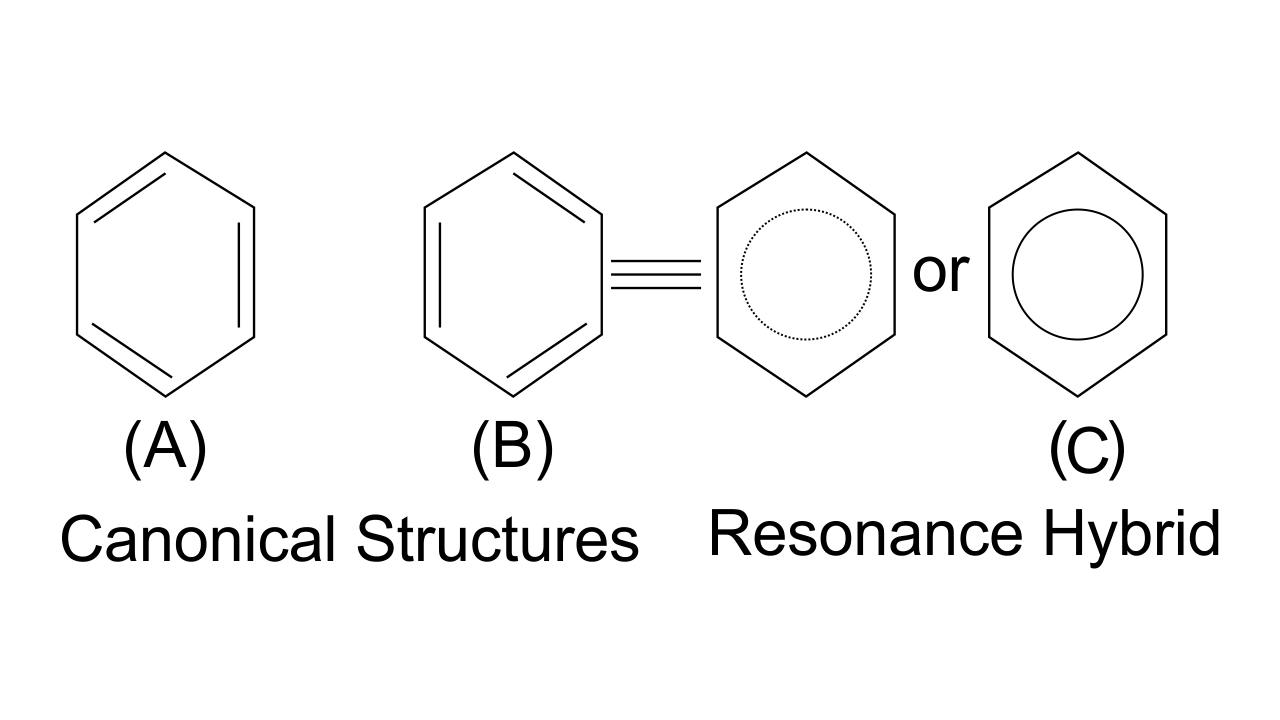57. Assertion (A): Pent- 1- ene and pent- 2- ene are position isomers. Reason (R): Position isomers differ in the position of functional group or substituent.

(i) Both A and R are correct, and R is the correct explanation of A.

(ii) Both A and R are correct, but R is not the correct explanation of A.

(iii) Both A and R are not correct. (iv) A is not correct but R is correct.

Ans: Option (i) i.e.,  Both A and R are correct, and R is the correct explanation of A is the answer as when two or more compounds differ in the position of substituent atom or functional group on the carbon skeleton, they are known as position isomers and this phenomenon is termed as position isomerism. Pent-2-ene and pent-l-ene are position isomers because they differ in the position of the double bond.

58. Assertion (A): All the carbon atoms in ${{\mathbf{H}}_{\mathbf{2}}}\mathbf{C}=\mathbf{C}=\mathbf{C}{{\mathbf{H}}_{\mathbf{2}}}$ are $\mathbf{s}{{\mathbf{p}}^{\mathbf{2}}}$ hybridized

Reason (R): In this molecule, all the carbon atoms are attached to each other by double bonds.

(i) Both A and R are correct, and R is the correct explanation of A.

(ii) Both A and R are correct, but R is not the correct explanation of A.

(iii) Both A and R are not correct.

(iv) A is not correct but R is correct.

Ans: Option (iv) i.e., A is not correct, but R is correct is the answer since hybridization of C can be found out by counting σ bonds and π bonds present on C atom. This can be shown as-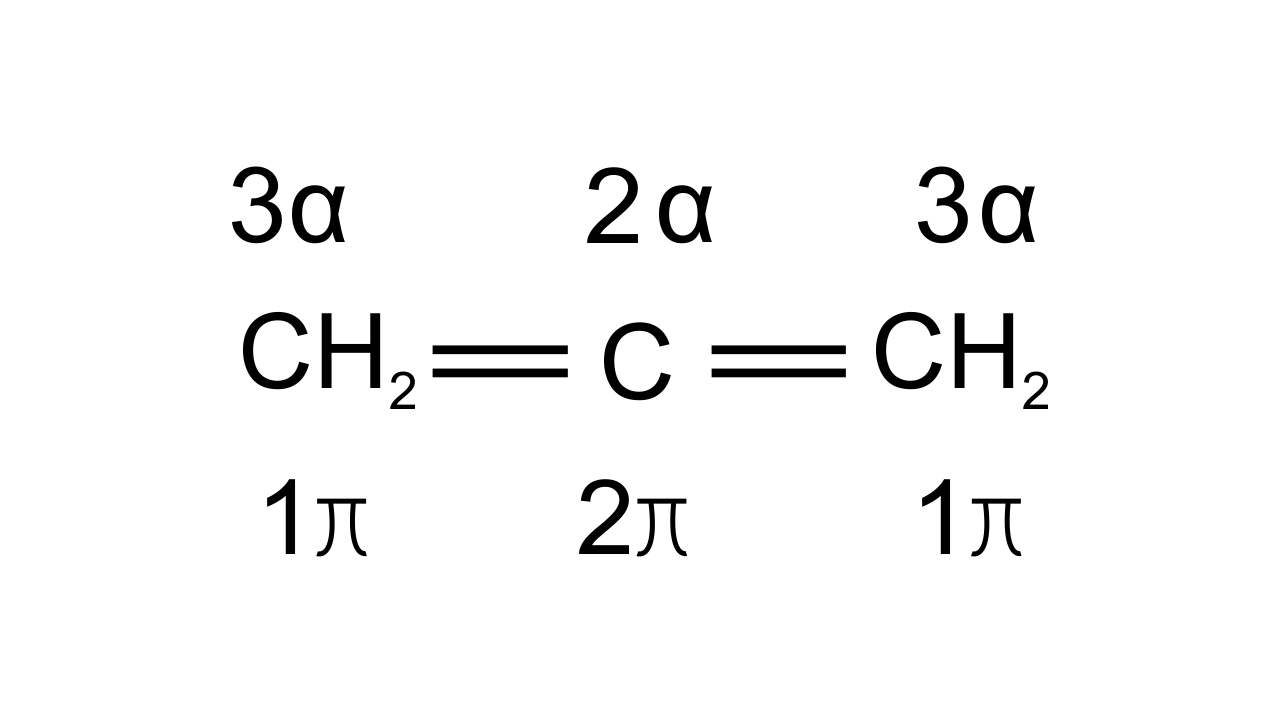If C has 3σ bonds then it is $\text{s}{{\text{p}}^{\text{2}}}$hybridized. If C has 2σ bonds then it is sp hybridized.

59. Assertion (A): Sulphur present in an organic compound can be estimated quantitatively by Carious method.

Reason (R): Sulphur is separated easily from other atoms in the molecule and gets precipitated as light-yellow solid.

(i) Both A and R are correct, and R is the correct explanation of A.

(ii) Both A and R are correct, but R is not the correct explanation of A.

(iii) Both A and R are not correct.

(iv) A is not correct but R is correct.

Ans: Option (iii) i.e., Both A and R are not correct is the answer since sulphur is estimated by Carius method in the form of white precipitate of $\text{BaS}{{\text{O}}_{\text{4}}}$ on heating with fuming and $\text{BaC}{{\text{l}}_{\text{2}}}$. If light yellow solid is obtained means impurities are present. It is filtered, washed and then dried to get pure $\text{BaS}{{\text{O}}_{\text{4}}}$ .

60. Assertion (A): Components of a mixture of red and blue inks can be separated by distributing the components between stationary and mobile phases in paper chromatography.

Reason (R): The coloured components of inks migrate at different rates because paper selectively retains different components according to the difference in their partition between the two phases.

(i) Both A and R are correct, and R is the correct explanation of A.

(ii) Both A and R are correct, but R is not the correct explanation of A.

(iii) Both A and R are not correct. (iv) A is not correct but R is correct.

Ans: Option (i) i.e.,  Both A and R are correct, and R is the correct explanation of A is the answer since in paper chromatography, a special quality of paper known as chromatography paper is used. Chromatography paper contains water molecules trapped in it which acts as the stationary phase. A strip of chromatography paper spotted at the base with the solution of the mixture is suspended in a suitable solvent or a mixture of solvents which acts as the mobile phase. The solvent rises up the paper by capillary action and flows over the spot. The paper selectively retains different components according to their differing partition in the two phases. During this process the paper strip so developed is known as a chromatogram and the spots of the separated coloured compounds are visible at different heights from the position of the initial spot on the chromatogram.

61. What is meant by hybridization? Compound ${{\mathbf{H}}_{\mathbf{2}}}\mathbf{C}=\mathbf{C}=\mathbf{C}{{\mathbf{H}}_{\mathbf{2}}}$ contains sp or $\mathbf{s}{{\mathbf{p}}^{\mathbf{2}}}$ hybridized carbon atoms. Will it be a planar molecule?

Ans: The atomic orbitals combine to give rise to a new set of equivalent orbitals called hybrid orbitals and are used in bond formation. This phenomenon is known as hybridization which can be defined as the process of intermixing of the orbitals of slightly different energies so as to redistribute their energies, resulting in the formation of new sets of orbitals having equivalent energies and shape. In ${{\text{H}}_{\text{2}}}\text{C=C=C}{{\text{H}}_{\text{2}}}$ (allene) carbon atom l and 3 are $\text{s}{{\text{p}}^{\text{2}}}$ hybridized as each one has 3σ bonds while carbon atom 2 has 2σ bonds so it is sp hybridized while the allene molecule as a whole is found to be non-planar.

62. Benzoic acid is an organic compound. Its crude sample can be purified by crystallization from hot water. What characteristic differences in the properties of benzoic acid and the impurity make this process of purification suitable?

Ans: Impurities present in benzoic acid are either insoluble in water or more soluble in water to such an extent that they remain in solution. Benzoic acid crystallizes from mother liquor.

63. Two liquids (A) and (B) can be separated by the method of fractional distillation. The boiling point of liquid (A) is less than boiling point of liquid (B). Which of the liquids do you expect to come out first in the distillate? Explain.

Ans: When the difference in boiling points of two liquids is not much then simple distillation cannot be used to separate them. Vapours of such liquids are formed within the same temperature range and are condensed simultaneously. The technique of fractional distillation is used in such cases. In this technique, vapours of a liquid mixture are passed through a fractionating column before condensation. The fractionating column is fitted over the mouth of the round bottom flask. The liquid (A) with low boiling point will distill first.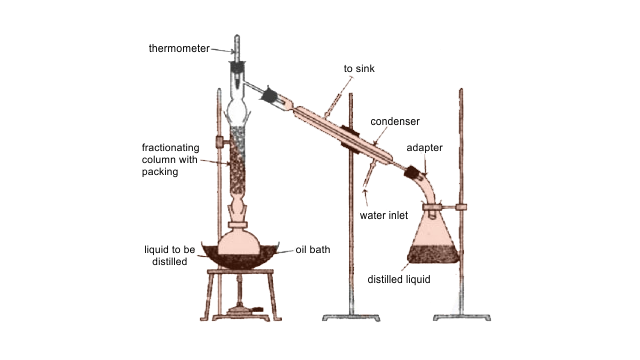64. You have a mixture of three liquids A, B and C. There is a large difference in the boiling points of A and rest of the two liquids i.e., B and C. Boiling point of liquids B and C are quite close. Liquid A boils at a higher temperature than B and C and boiling point of B is lower than C. How will you separate the components of the mixture? Draw a diagram showing set up of the apparatus for the process.

Ans: Liquids which have different boiling points vaporize at different temperatures. The vapours are cooled and then liquids so formed are collected separately. Liquid A can be separated from B and C due to the large difference in boiling points. Liquid B and Liquid C have boiling points very close to each other and cannot be separated by simple distillation and are separated by fractional distillation. Liquid B distilled first since the order of boiling points of A, B and C is as follows: B < C < A.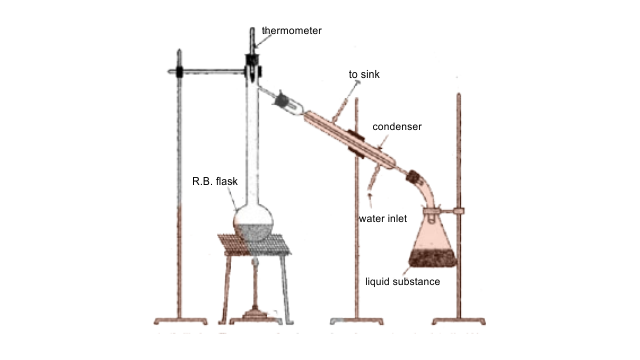65. Draw a diagram of bubble plate type fractionating column. When do we require such type of a column for separating two liquids. Explain the principle involved in the separation of components of a mixture of liquids by using fractionating column. What industrial applications does this process have?

Ans: Whenever the difference in the boiling points of two liquids is not much than simple distillation technique cannot be used to separate them. The vapours of such liquids are formed within the same temperature range and are condensed simultaneously and hence the technique of fractional distillation is used in such cases. In this technique, vapours of a liquid mixture are passed through a fractionating column before condensation. The fractionating column is fitted over the mouth of the round bottomed flask. The vapours of the liquid with higher boiling point condense before the vapours of the liquid with lower boiling point. The vapours rising up in the fractionating column become richer in more volatile components and by the time the vapours reach to the top of the fractionating column, these become rich in more volatile components.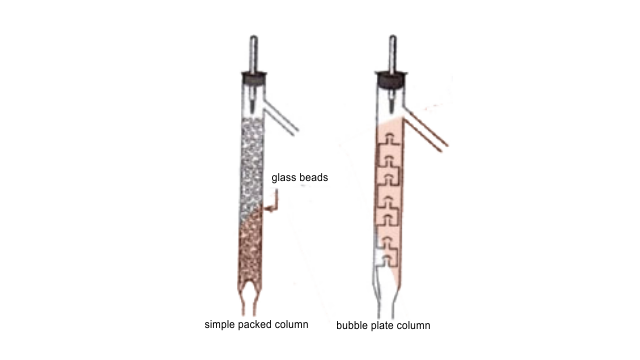66. A liquid with high boiling point decomposes on simple distillation but it can be steam distilled for its purification. Explain how is it possible?

Ans: Steam distillation is a special type of separation technique for temperature sensitive materials such as natural organic compounds. Few organic compounds tend to decompose at higher temperatures and normal distillation does not fit for this purpose. So, water is added to the apparatus and the temperature of the compounds is depressed, evaporating them at lower temperature. Once the distillation is accomplished, the vapours are condensed and  there occurs,  the separation of the constituents at ease.

### Summary of Chapter 12 Organic Chemistry of Chemistry of Class 11

In Chemistry, compounds were classified mainly into two categories: Organic Compounds and Inorganic compounds.

Compounds obtained from non-living matter are labeled as Inorganic compounds.

And the compounds obtained from living sources such as plants and animal sources are called Organic Compounds. Later people realized that all the Organic Compounds contain carbons. Hence they are also called Carbon compounds

Organic Chemistry is just the part of Chemistry that studies these carbon compounds. But this definition seems off as there are many compounds that do contain carbon but are not considered organic compounds. For example Carbon monoxide (CO), Carbon Dioxide (CO2), carbon disulphide, and calcium carbonate, all these compounds contain carbon atoms but are considered Inorganic Compounds. Studies have shown that inorganic compounds, carbon atoms are in some sort of relationship with hydrogen. Thus the modern definition of organic chemistry is the branch of chemistry that deals with the chemistry of Hydrocarbons and their derivatives.

## FAQs on NCERT Exemplar for Class 11 Chemistry Chapter-12 (Book Solutions)

1. What are the rules for IUPAC nomenclature of branched-chain saturated hydrocarbons (Alkanes) as taught in the Class 11 Chemistry chapter?

Rules for IUPAC nomenclature of branched-chain saturated hydrocarbons:

• Longest Chain Rule: Select the longest continuous chain of carbon atoms to give the name of the parent hydrocarbon.

• Position of Substituent: Number of carbons atoms of the parent chain (as 1, 2, 3 … etc) starting from the end which gives the lower number to the carbon atoms carrying the substituents.

• The Lowest set of Locants: When more than 1 substituent is present, the end of the parent chain which gives the lowest set of locants is preferred.

• Presence of the same Substituent Multiple times: If the same substituent or side chain is present multiple times, then the prefixes di (for 2), Tri (for 3), tetra (fro 4), Penta ...etc.are attached to the name of the substituent.

2. What is the difference between Electrophiles and Nucleophiles according to Chapter 12 of Class 11 Chemistry?

Here are the differences between Electrophiles and Nucleophiles:

• Electrophiles are deficient in electrons, while Nucleophiles have an excess of them.

• Electrophiles behave as Lewis acids, while Nucleophiles as Lewis bases.

• Electrophiles accept a pair of electrons from the substrate (as they are deficient in electrons), while the other (Nucleophiles) donate their pair of electrons to the substrate, as they are rich in electrons.

• Both of them might be neutral in charge, but electrophiles can be positively charged and Nucleophiles can be Negatively charged.

3. What are the major topics and sub-topics from Chapter 12 Organic Chemistry in Class 11?

These are some the major topics and subtopics from that are important from exam perspective:

1. General Introduction

2. Tetravalence Of Carbon: Shapes of Organic Compounds

3. Structural Representations Of Organic Compounds

4. Classification of Organic Compounds

5. Nomenclature of Organic Compounds

1. The IUPAC System Of Nomenclature

2. Iupac Nomenclature Of Alkanes

3. Nomenclature of Organic Compounds Having Functional Group(S)

4. Nomenclature of Substituted Benzene Compounds

6. Isomerism ( structural and stereoisomerism)

7. Fundamental Concepts In Organic Reaction Mechanism

8. Methods Of Purification of Organic Compounds

1. Sublimation

2. Crystallization

3. Distillation

4. Differential Extraction

5. Chromatography

6. Qualitative Analysis of Organic Compounds

9. Quantitative Analysis of various elements found in Organic compounds namely carbon, hydrogen, nitrogen, halogens.

4. What are Isomers as mentioned in Chapter 12 Organic Chemistry?

Compounds having the same molecular formula but have different physical and chemical properties are called Isomers. The phenomenon has been named Isomerism.

Isomerism is common of two types:

1. Structural Isomerism

2. Stereo Isomerism

Structural Isomers: Compounds having the same molecular formula but with different structures or with different arrangements of atoms or groups of atoms.

Stereoisomers: Compounds with the same molecular formula but the different relative arrangement of atoms or groups of them.

5. How to Download the Solution to NCERT exemplar questions of Class 11 Chapter 12 Organic Chemistry?

Students can Download the NCERT exemplar solutions for Class 11 Chapter 12 Organic Chemistry by visiting the official website of Vedantu. They will be to find these solutions in the study material section on the top bar of the website.

Students will have to enter their Email address, to the entered Email address, the required PDF file of the NCERT Exemplar solutions will be sent. Vedantu provides these solutions free of charge to students preparing for the exams, students can download them for free and can access them at any time and anywhere they want.